# JP4494495B2 - Optical multiplexing / demultiplexing circuit with phase generation function - Google Patents

## Info

Publication number
JP4494495B2
JP4494495B2 JP2008146281A JP2008146281A JP4494495B2 JP 4494495 B2 JP4494495 B2 JP 4494495B2 JP 2008146281 A JP2008146281 A JP 2008146281A JP 2008146281 A JP2008146281 A JP 2008146281A JP 4494495 B2 JP4494495 B2 JP 4494495B2
Authority
JP
Japan
Prior art keywords
optical
phase
path length
multiplexing
length difference
Prior art date
Legal status (The legal status is an assumption and is not a legal conclusion. Google has not performed a legal analysis and makes no representation as to the accuracy of the status listed.)
Active
Application number
JP2008146281A
Other languages
Japanese (ja)
Other versions
JP2008209955A (en
Inventor

Original Assignee

Priority date (The priority date is an assumption and is not a legal conclusion. Google has not performed a legal analysis and makes no representation as to the accuracy of the date listed.)
Filing date
Publication date
Priority to JP2002354640 priority Critical
Application filed by 日本電信電話株式会社 filed Critical 日本電信電話株式会社
Priority to JP2008146281A priority patent/JP4494495B2/en
Publication of JP2008209955A publication Critical patent/JP2008209955A/en
Application granted granted Critical
Publication of JP4494495B2 publication Critical patent/JP4494495B2/en
Active legal-status Critical Current
Anticipated expiration legal-status Critical

• 230000001808 coupling Effects 0.000 claims description 92
• 238000010168 coupling process Methods 0.000 claims description 92
• 238000005859 coupling reaction Methods 0.000 claims description 92
• 238000005365 production Methods 0.000 description 16
• 238000005457 optimization Methods 0.000 description 11
• 239000000463 materials Substances 0.000 description 10
• 230000000694 effects Effects 0.000 description 8
• 238000000206 photolithography Methods 0.000 description 8
• 238000001020 plasma etching Methods 0.000 description 8
• 238000000151 deposition Methods 0.000 description 3
• 238000004364 calculation methods Methods 0.000 description 2
• 238000005253 cladding Methods 0.000 description 2
• 238000000034 methods Methods 0.000 description 2
• 238000005516 engineering processes Methods 0.000 description 1
• 239000010408 films Substances 0.000 description 1
• 238000004519 manufacturing process Methods 0.000 description 1

## Images

•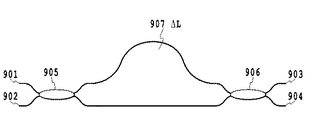•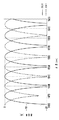•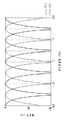•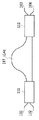•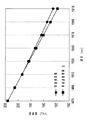•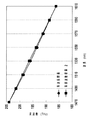•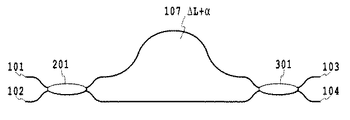•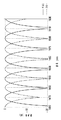•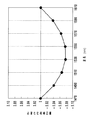•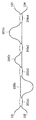•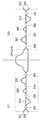•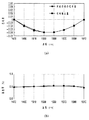•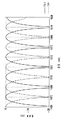•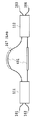•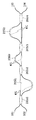•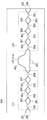•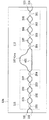•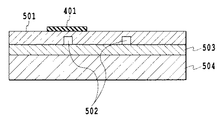•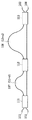•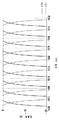•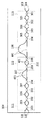•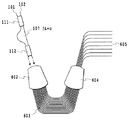•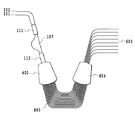•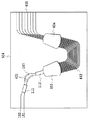•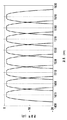•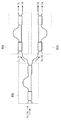•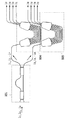•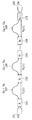•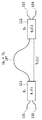•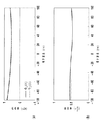•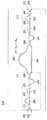•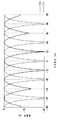•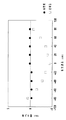•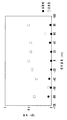•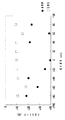•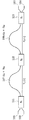••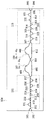•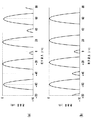•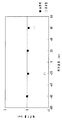•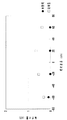•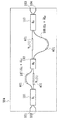•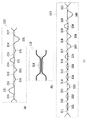•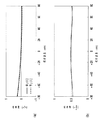•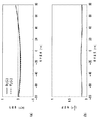•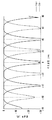•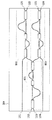•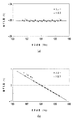•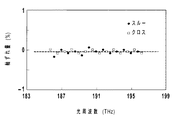•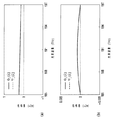•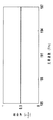•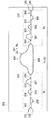•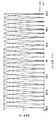••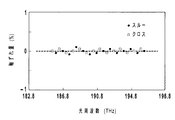•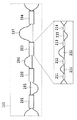•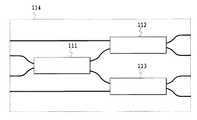•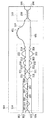•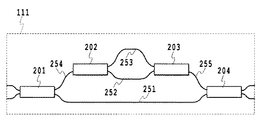•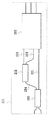•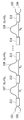•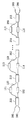•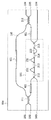•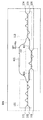•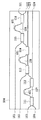•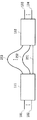•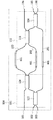•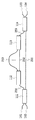•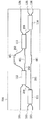••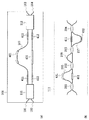•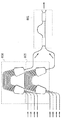•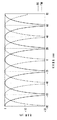•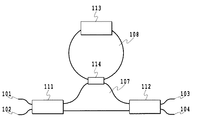•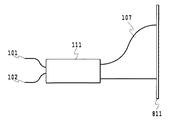•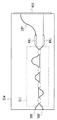•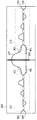•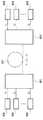•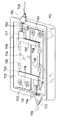•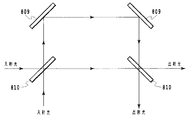## Classifications

• GPHYSICS
• G02OPTICS
• G02BOPTICAL ELEMENTS, SYSTEMS, OR APPARATUS
• G02B6/00Light guides
• G02B6/10Light guides of the optical waveguide type
• G02B6/12Light guides of the optical waveguide type of the integrated circuit kind
• G02B6/12007Light guides of the optical waveguide type of the integrated circuit kind forming wavelength selective elements, e.g. multiplexer, demultiplexer
• G02B6/12009Light guides of the optical waveguide type of the integrated circuit kind forming wavelength selective elements, e.g. multiplexer, demultiplexer comprising arrayed waveguide grating [AWG] devices, i.e. with a phased array of waveguides
• G02B6/12019Light guides of the optical waveguide type of the integrated circuit kind forming wavelength selective elements, e.g. multiplexer, demultiplexer comprising arrayed waveguide grating [AWG] devices, i.e. with a phased array of waveguides characterised by the optical interconnection to or from the AWG devices, e.g. integration or coupling with lasers or photodiodes
• GPHYSICS
• G02OPTICS
• G02BOPTICAL ELEMENTS, SYSTEMS, OR APPARATUS
• G02B6/00Light guides
• G02B6/10Light guides of the optical waveguide type
• G02B6/12Light guides of the optical waveguide type of the integrated circuit kind
• G02B6/12007Light guides of the optical waveguide type of the integrated circuit kind forming wavelength selective elements, e.g. multiplexer, demultiplexer
• GPHYSICS
• G02OPTICS
• G02BOPTICAL ELEMENTS, SYSTEMS, OR APPARATUS
• G02B6/00Light guides
• G02B6/10Light guides of the optical waveguide type
• G02B6/12Light guides of the optical waveguide type of the integrated circuit kind
• G02B6/12007Light guides of the optical waveguide type of the integrated circuit kind forming wavelength selective elements, e.g. multiplexer, demultiplexer
• G02B6/12009Light guides of the optical waveguide type of the integrated circuit kind forming wavelength selective elements, e.g. multiplexer, demultiplexer comprising arrayed waveguide grating [AWG] devices, i.e. with a phased array of waveguides
• G02B6/12016Light guides of the optical waveguide type of the integrated circuit kind forming wavelength selective elements, e.g. multiplexer, demultiplexer comprising arrayed waveguide grating [AWG] devices, i.e. with a phased array of waveguides characterised by the input or output waveguides, e.g. tapered waveguide ends, coupled together pairs of output waveguides
• GPHYSICS
• G02OPTICS
• G02BOPTICAL ELEMENTS, SYSTEMS, OR APPARATUS
• G02B6/00Light guides
• G02B6/10Light guides of the optical waveguide type
• G02B6/12Light guides of the optical waveguide type of the integrated circuit kind
• G02B6/12007Light guides of the optical waveguide type of the integrated circuit kind forming wavelength selective elements, e.g. multiplexer, demultiplexer
• G02B6/12009Light guides of the optical waveguide type of the integrated circuit kind forming wavelength selective elements, e.g. multiplexer, demultiplexer comprising arrayed waveguide grating [AWG] devices, i.e. with a phased array of waveguides
• G02B6/12019Light guides of the optical waveguide type of the integrated circuit kind forming wavelength selective elements, e.g. multiplexer, demultiplexer comprising arrayed waveguide grating [AWG] devices, i.e. with a phased array of waveguides characterised by the optical interconnection to or from the AWG devices, e.g. integration or coupling with lasers or photodiodes
• G02B6/12021Comprising cascaded AWG devices; AWG multipass configuration; Plural AWG devices integrated on a single chip
• GPHYSICS
• G02OPTICS
• G02BOPTICAL ELEMENTS, SYSTEMS, OR APPARATUS
• G02B6/00Light guides
• G02B6/10Light guides of the optical waveguide type
• G02B6/12Light guides of the optical waveguide type of the integrated circuit kind
• G02B6/12007Light guides of the optical waveguide type of the integrated circuit kind forming wavelength selective elements, e.g. multiplexer, demultiplexer
• G02B6/12009Light guides of the optical waveguide type of the integrated circuit kind forming wavelength selective elements, e.g. multiplexer, demultiplexer comprising arrayed waveguide grating [AWG] devices, i.e. with a phased array of waveguides
• G02B6/12023Light guides of the optical waveguide type of the integrated circuit kind forming wavelength selective elements, e.g. multiplexer, demultiplexer comprising arrayed waveguide grating [AWG] devices, i.e. with a phased array of waveguides characterised by means for reducing the polarisation dependence, e.g. reduced birefringence
• GPHYSICS
• G02OPTICS
• G02BOPTICAL ELEMENTS, SYSTEMS, OR APPARATUS
• G02B6/00Light guides
• G02B6/24Coupling light guides
• G02B6/26Optical coupling means
• G02B6/28Optical coupling means having data bus means, i.e. plural waveguides interconnected and providing an inherently bidirectional system by mixing and splitting signals
• G02B6/293Optical coupling means having data bus means, i.e. plural waveguides interconnected and providing an inherently bidirectional system by mixing and splitting signals with wavelength selective means
• G02B6/29346Optical coupling means having data bus means, i.e. plural waveguides interconnected and providing an inherently bidirectional system by mixing and splitting signals with wavelength selective means operating by wave or beam interference
• G02B6/2935Mach-Zehnder configuration, i.e. comprising separate splitting and combining means
• G02B6/29352Mach-Zehnder configuration, i.e. comprising separate splitting and combining means in a light guide
• G02B6/29353Mach-Zehnder configuration, i.e. comprising separate splitting and combining means in a light guide with a wavelength selective element in at least one light guide interferometer arm, e.g. grating, interference filter, resonator
• GPHYSICS
• G02OPTICS
• G02BOPTICAL ELEMENTS, SYSTEMS, OR APPARATUS
• G02B6/00Light guides
• G02B6/24Coupling light guides
• G02B6/26Optical coupling means
• G02B6/28Optical coupling means having data bus means, i.e. plural waveguides interconnected and providing an inherently bidirectional system by mixing and splitting signals
• G02B6/293Optical coupling means having data bus means, i.e. plural waveguides interconnected and providing an inherently bidirectional system by mixing and splitting signals with wavelength selective means
• G02B6/29346Optical coupling means having data bus means, i.e. plural waveguides interconnected and providing an inherently bidirectional system by mixing and splitting signals with wavelength selective means operating by wave or beam interference
• G02B6/2935Mach-Zehnder configuration, i.e. comprising separate splitting and combining means
• G02B6/29352Mach-Zehnder configuration, i.e. comprising separate splitting and combining means in a light guide
• GPHYSICS
• G02OPTICS
• G02BOPTICAL ELEMENTS, SYSTEMS, OR APPARATUS
• G02B6/00Light guides
• G02B6/24Coupling light guides
• G02B6/26Optical coupling means
• G02B6/28Optical coupling means having data bus means, i.e. plural waveguides interconnected and providing an inherently bidirectional system by mixing and splitting signals
• G02B6/293Optical coupling means having data bus means, i.e. plural waveguides interconnected and providing an inherently bidirectional system by mixing and splitting signals with wavelength selective means
• G02B6/29379Optical coupling means having data bus means, i.e. plural waveguides interconnected and providing an inherently bidirectional system by mixing and splitting signals with wavelength selective means characterised by the function or use of the complete device
• G02B6/2938Optical coupling means having data bus means, i.e. plural waveguides interconnected and providing an inherently bidirectional system by mixing and splitting signals with wavelength selective means characterised by the function or use of the complete device for multiplexing or demultiplexing, i.e. combining or separating wavelengths, e.g. 1xN, NxM

## Description

The present invention relates to an optical multiplexing / demultiplexing circuit used in the field of optical communication, and more particularly to an optical multiplexing / demultiplexing circuit used for wavelength division multiplexing communication.

In recent years, Dense Wavelength Division Multiplexing (DWDM) systems have been developed, and optical devices having various functions that are indispensable for WDM systems such as wavelength multiplexers / demultiplexers, optical filters, and optical switches have been developed. Has been developed.

Examples of such optical devices include an arrayed waveguide grating, a lattice filter (see, for example, Non-Patent Document 1), an asymmetric Mach-Zehnder interferometer (for example, see Non-Patent Document 2), and a symmetric Mach-Zehnder interferometer ( For example, a non-patent document 3), a multistage Mach-Zehnder interferometer (for example, refer to non-patent document 4), a transversal filter (for example, refer to non-patent document 5), and the like have been reported.

More recently, demand for optical devices for CWDM (Coarse Wavelength Division Multiplexing) systems (see, for example, Non-Patent Document 6) used particularly in metro networks has been increasing.

The wavelength grid of such a CWDM system has an equal wavelength interval, and the interval is 20 nm. Therefore, it is required to make the transmission band of an optical device for a CWDM system so as to have equal wavelength intervals.

However, a conventional optical multiplexing / demultiplexing circuit such as a Mach-Zehnder interference circuit has an equal frequency interval, and the wavelength characteristics are not periodic on the equal wavelength axis. Therefore, the insertion loss, transmission bandwidth, and extinction ratio vary depending on the wavelength and cannot be used for the CWDM system.

As a specific example, FIG. 1 shows a conventional Mach-Zehnder interferometer. The Mach-Zehnder interferometer (see, for example, Non-Patent Document 7) includes two optical couplers 905 and 906, an optical path length difference providing unit 907 sandwiched between the two optical couplers, and an optical coupler 905. , 906 and two input / output optical waveguides connected to 906, respectively. The optical couplers 905 and 906 use directional couplers, and the coupling rate is set to 50%.

The Mach-Zehnder interferometer is a multiplexing / demultiplexing circuit with equal frequency intervals as described below. The two light output intensities of the Mach-Zehnder interferometer are

It is expressed.

Where A 0 is the intensity of light incident from one input port, and ξ is the phase of the optical path length difference providing means.

Here, from the relationship f = c / λ, ξ is

It is.

Where n is the refractive index, ΔL is the optical path length difference, f is the frequency, c is the speed of light, λ is the wavelength, and m is an integer.

Using the cosine function and the square of the sine function as a function of period π, the frequency interval

Since it is a constant, it can be seen that the Mach-Zehnder interferometer is a multiplexing / demultiplexing circuit with equal frequency intervals.

FIG. 2 shows an optical path length difference that gives a frequency interval such that the center wavelength of the Mach-Zehnder interferometer is 1470 nm and the demultiplexing interval between the through port and the cross port in the vicinity is 20 nm, that is, the optical path length difference providing means. The transmission characteristics when the optical path length difference is set to 55.9 μm are shown. Here, the horizontal axis is the wavelength, and wavelength grids are arranged at equal intervals. The solid line is the transmission characteristic output from the cross port, and the broken line is the transmission characteristic output from the through port.

For comparison, the transmission characteristics when the horizontal axis is the optical frequency are shown in FIG. The transmission characteristics of the Mach-Zehnder interferometer are equal frequency intervals as shown in FIG. 3, and the wavelength intervals are not equal. In the vicinity of 1470 nm, the demultiplexing interval between the through port and the cross port is 20 nm and is placed on the wavelength grid, but it is clear that the wavelength interval increases as the wavelength increases from 1470 nm to the longer wavelength side, and the distance from the wavelength grid increases. Therefore, such an optical device is not suitable for use as an optical device for a CWDM system because its insertion loss, transmission bandwidth, and extinction ratio vary significantly depending on the wavelength on the wavelength grid.

That is, since the interference circuit such as the Mach-Zehnder interference circuit of the conventional configuration is not at the same wavelength interval, the transmission band shifts from the grid on the wavelength axis as the distance from the center wavelength increases, and the insertion loss varies significantly depending on the wavelength. there were.

The conventional optical multiplexing / demultiplexing circuit has an equal period characteristic in the optical frequency region, but its transmission characteristic can be characterized by the optical frequency interval and the central optical frequency. However, in the conventional optical multiplexing / demultiplexing circuit, the design parameter that can be freely set related to the optical frequency interval and the center optical frequency is only the optical optical path length difference of the optical path length difference providing means. While there are two parameters that need to be set, since only one parameter can be freely set, the optical frequency interval and the center optical frequency cannot be set simultaneously.

Therefore, in principle, the optical multiplexing / demultiplexing circuit having the equal frequency interval cannot be set either in the optical frequency interval or the central optical frequency, and when used in the optical frequency region, the transmission characteristics are deteriorated as a result. There was a problem.

In order to solve the above-mentioned problems, the inventors provide a mechanism in which the optical path length difference ΔL changes for each grid so that the transmission band is on the grid with the equal wavelength interval, and the center wavelength is set to the grid. I came up with the idea that it would be better to match. The optical path length difference ΔL is usually given by the optical path length difference providing means, but it has been noted that the optical path length difference ΔL can also be given by the phase difference of the output of the optical multiplexing / demultiplexing means constituting the interferometer.

For example, if a phase difference of 1 period π is given between two output ports of a Mach-Zehnder interferometer, the optical path length can be changed by ½ wavelength. Therefore, if an optical multiplexing / demultiplexing means whose output phase difference has wavelength dependence is realized and applied to the optical path length difference providing means of the Mach-Zehnder interferometer, the optical path length difference ΔL can be changed for each different wavelength. become.

However, in conventional optical multiplexing / demultiplexing means such as directional couplers and multimode interferometer type couplers used in the prior art, the phase difference of the output is constant, so that it depends on the wavelength (frequency). There was no phase difference, and wavelength (frequency) dependence due to the contribution of the optical multiplexing / demultiplexing means could not be given to the optical path length difference ΔL.

JP-A-11-109147 (FIG. 6) JP-A-8-069021 (FIGS. 6 and 7) JP 2003-057457 A M. Oguma et al., "Passband-width broadening design for WDM filter with lattice-form interleave filter and arrayed-waveguide gratings" IEEE Photonics Technology Letters, Vol. 14, pp. 328-330 (2002). De Merlier et al., "All-optical 2R regeneration based on integrated asymmetric Mach-Zehnder interferometer incorporating MMI-SOA" Electronics Letters, Vol. 38, pp. 238-239 (2002). Y. Hashizume et al., "Integrated polarization beam splitter using waveguide birefringence dependence on waveguide core width" Electronics Letters, Vol. 37, pp. 1517-1518 (2001). K. Suzuki et al., "PLC-based dynamic gain equaliser consisting of integrated Mach-Zehnder interferometers with C- and L-band equalising range" Electronics Letters, Vol. 38, pp. 1030-1031 (2002). T. Mizuno et al., "Dispersionless interleave filter based on transversal form optical filter" Electronics Letters, Vol. 38, pp. 1121-1122 (2002). R.R.Patel et al., "Multi-mode fiber coarse WDM grating router using broadband add / drop filters for wavelength re-use" LEOS'99 12th Annual Meetmg Vol. 2, pp. 826-827 (1999). K. Okamoto, "Fundamentals of optical waveguldes" Academic Press, pp. 159-161 (2000). M. Oguma et al., "Flat-passband interleave filter with 200 GHZ channel spacing based on planar lightwave circuit-type lattice structure," Electronics Letters, Vol. 36, pp. 1299-1300 (2000). C. R. Doerr et al., "Compact and low-loss integrated flat-top passband demux" 27th European Conference on Optical Communication ECOC 'O1 Vol. 6, pp. 24-25 (2001). M. Oguma et al., OFC 2002 TuK3 (2002). M. Kawachi "Silica Waveguides on Silicon and Their Application to Integrated-Optic Components", Opt. Quantum Electron., Vol.22, pp.391-416 (1990).

The present invention provides an optical multiplexing / demultiplexing means having a phase generation function for giving a different phase difference to light having a wavelength (frequency) in a transmission band of a circuit, in which an output phase difference has wavelength (frequency) dependence. An object of the present invention is to provide an optical multiplexing / demultiplexing circuit having equal optical frequency intervals in which a transmission band rides on an optical frequency grid.

Further, by using the phase generation means of the present invention, an optical frequency division that allows an optical frequency dependent phase to act on the optical multiplexing / demultiplexing circuit, and the optical frequency interval and the central optical frequency can be set to arbitrary values. An object is to provide a wave circuit.

In order to achieve the above-described object, the present invention provides an optical multiplexing / demultiplexing circuit that multiplexes / demultiplexes a plurality of lights in an entire band composed of a plurality of transmission bands, and has at least two inputs and outputs. An optical multiplexing / demultiplexing unit; and an optical path length difference providing unit connected between the at least two optical multiplexing / demultiplexing units, wherein at least one of the optical multiplexing / demultiplexing units functions as a phase generating unit, and the phase generating unit Generates a phase φ having an optical frequency dependency defined as a phase difference between two lights output from two output terminals in the output unit, and is generated by one or more of the phase generation means A phase Φ represented by a function of optical frequency and having optical frequency dependency generated in the optical path length difference providing means is a constant frequency interval of each central optical frequency of the adjacent transmission band and a central optical frequency of the entire band. Desired An optical multiplexing and demultiplexing circuit and setting the same time value.

Preferably, the transmission characteristics in the entire band are such that N is the order of the optical multiplexing / demultiplexing circuit, xq is the expansion coefficient, f is the optical frequency , and Ψ (f) is the center optical frequency between adjacent transmission bands on the optical frequency domain grid. The target phase having optical frequency dependency necessary for setting the interval, ΔL ′ (= ΔL + δL), the optical path length difference of the optical path length difference adding means including the wavelength dependency of the refractive index, and ΔL are optical When the optical path length difference, δL, is defined as a micro optical optical path length difference, it is expressed by the following equation.

Then, the phase Φ having the optical frequency dependency generated by the phase generating means may be equal to the target phase ψ having the optical frequency dependency.

More preferably, the phase Φ having optical frequency dependency is given by a function of the optical frequency (f) in the entire band of the optical multiplexing / demultiplexing circuit, and the function is a polynomial having a quadratic function or more, The target phase Ψ having frequency dependence is expressed as follows: Δf is the optical frequency interval of the multiplexing / demultiplexing circuit on the optical frequency domain grid, fc is the center optical frequency of the transmission band located near the center of the entire band of the optical multiplexing / demultiplexing circuit, If m is an integer, it can be expressed by the following equation.

The phase generation means includes (M + 1) (M is an integer of 2 or more) optical couplers;
Said optical coupler M optical path length difference imparting portion disposed between the adjacent and are composed of a phase having the optical frequency dependent amplitude coupling rate and the optical path length of said optical coupler It can also be generated by optimizing the optical path length difference of the difference providing unit.

In addition, the phase generation unit includes (M + 1) (M is an integer of 2 or more) optical couplers and M optical path length difference providing units arranged between the adjacent optical couplers. and which, as the phase Φ with the optical frequency dependence is made equal to the target phase having an optical frequency dependence [psi, optical Tekihikariro amplitude coupling rate and the optical path length difference imparting portion of the optical coupler Each of the length difference and the optical path length difference ΔL added to the optical path length difference providing means provided in the optical multiplexing / demultiplexing circuit may be set.

The optical multiplexing / demultiplexing circuit is an optical interference circuit, and the optical interference circuit is provided between (N + 1) (N is an integer of 1 or more) optical multiplexing / demultiplexing means and the adjacent optical multiplexing / demultiplexing means. It can be configured by connecting the N optical path length difference providing means arranged.

In another aspect of the present invention, the optical multiplexing / demultiplexing circuit includes two optical multiplexing / demultiplexing units, the optical path length difference providing unit disposed between the two optical multiplexing / demultiplexing units, and the optical multiplexing / demultiplexing unit. A Mach-Zehnder interference circuit comprising at least one input waveguide connected to one of the optical waveguides and a plurality of output waveguides connected to the other of the optical multiplexing / demultiplexing means, wherein the two optical multiplexing / demultiplexing means The phase generation optical coupler is a phase generation optical coupler which is arranged symmetrically with respect to the intermediate point of the Mach-Zehnder interference circuit, and both of the two optical multiplexing / demultiplexing means include phase generation means. May be configured by four optical couplers and three optical path length difference providing units respectively disposed between the adjacent optical couplers.

More preferably, the optical multiplexing / demultiplexing circuit is connected to one of the two optical multiplexing / demultiplexing means, the optical path length difference providing means disposed between the two optical multiplexing / demultiplexing means, and the optical multiplexing / demultiplexing means. A Mach-Zehnder interference circuit comprising at least one input waveguide and a plurality of output waveguides connected to the other of the optical multiplexing / demultiplexing means, wherein one of the two optical multiplexing / demultiplexing means is , A phase generation optical coupler including phase generation means, wherein the phase generation optical coupler is provided between (M + 1) (M is an integer of 2 or more) optical couplers and the adjacent optical couplers. You may be comprised by the M optical path length difference provision part arrange | positioned.

The optical multiplexing / demultiplexing circuit includes first to third optical multiplexing / demultiplexing units and two optical path length difference providing units arranged between the adjacent three optical multiplexing / demultiplexing units, A lattice filter comprising at least one input waveguide connected to the first optical multiplexing / demultiplexing means and at least one output waveguide connected to the third optical multiplexing / demultiplexing means; The first and third optical multiplexing / demultiplexing means are phase generating optical couplers having phase generating means, and the number of phase generating optical couplers is (M + 1) (M is an integer of 2 or more) optical couplers. And M optical path length difference providing units respectively disposed between the adjacent optical couplers.

Preferably, each of the optical multiplexing / demultiplexing circuits described above can be a transversal filter.

In yet another aspect of the present invention, the arrayed waveguide diffraction grating, the first slab waveguide connected to one end of the arrayed waveguide diffraction grating, and the other end of the arrayed waveguide diffraction grating are connected. A second slab waveguide; and an output waveguide connected to the second slab waveguide, wherein an output of the optical multiplexing / demultiplexing circuit is optically connected to the first slab waveguide. Also good.

Similarly, an arrayed waveguide diffraction grating, a first slab waveguide connected to one end of the arrayed waveguide grating, an input waveguide connected to the first slab waveguide, and the arrayed waveguide A second slab waveguide connected to the other end of the diffraction grating, and an output of the second slab waveguide may be optically connected to the optical multiplexing / demultiplexing circuit.

More preferably, the optical multiplexing / demultiplexing circuit includes two optical multiplexing / demultiplexing units, and the optical path length difference providing unit includes two optical delay lines disposed between the adjacent optical multiplexing / demultiplexing units. And at least one input waveguide is connected to one of the two optical multiplexing / demultiplexing means, and the other of the two optical multiplexing / demultiplexing means is connected to the first slab waveguide or the second of the arrayed waveguide diffraction grating. It may be connected to at least one of the slab waveguides.

Furthermore, one or more optical multiplexing / demultiplexing circuits described above may be connected to at least one output side of the optical multiplexing / demultiplexing circuit described above.

An optical multiplexing / demultiplexing circuit according to another embodiment of the present invention is an optical multiplexing / demultiplexing circuit that multiplexes / demultiplexes a plurality of lights in an entire band composed of a plurality of transmission bands, and has at least one input unit and an output unit. Two optical multiplexing / demultiplexing means, an optical path length difference providing means optically connected to the output part of the at least one optical multiplexing / demultiplexing means, and a reflector connected to the optical path length difference providing means, A reflection type optical multiplexing / demultiplexing circuit having a reflecting plate as a turning point of signal light, wherein at least one of the optical multiplexing / demultiplexing means functions as a phase generating means, and the phase generating means is provided in the output unit. A phase φ having an optical frequency dependency defined as a phase difference between two lights output from the two output ends, and expressed by a function of the optical frequency generated by one or more of the phase generating means; Occurs in the optical path length difference providing means Phase Φ is having an optical frequency dependence to, and sets the predetermined interval and the center optical frequency of the full bandwidth of the center optical frequency of the transmission band adjacent to the same time a desired value.

Preferably, the phase generation means includes (M + 1) (M is an integer of 2 or more) optical couplers and M optical path length difference providing units arranged between the adjacent optical couplers. The phase having the optical frequency dependency may be generated by optimizing the amplitude coupling ratio of the optical coupler and the optical optical path length difference of the optical path length difference providing unit.

In each of the above-described optical multiplexing / demultiplexing bottlenecks, it is preferable that the optical path length difference providing unit is provided with phase adjusting means or phase adjusted.

Further, in each of the optical multiplexing / demultiplexing bottleneck described above, it is preferable that the optical path length difference providing unit is provided with birefringence adjusting means or birefringence adjustment is performed.

Furthermore, in each of the above-described optical multiplexing / demultiplexing bottles, the optical multiplexing / demultiplexing circuit may be formed of a silica glass optical waveguide.

Furthermore, it is preferable that the optical multiplexing / demultiplexing circuit according to any one of the above is housed in a casing, and light is input / output to / from the optical multiplexing / demultiplexing circuit by an optical fiber held in the casing.

Still another embodiment of the present invention is a method of designing an optical multiplexing / demultiplexing circuit for multiplexing / demultiplexing a plurality of lights in an entire band composed of a plurality of transmission bands, wherein the optical multiplexing / demultiplexing circuit includes an input unit And at least two optical multiplexing / demultiplexing means, and an optical path length difference providing means connected between the at least two optical multiplexing / demultiplexing means, wherein at least one of the optical multiplexing / demultiplexing means generates a phase The phase generation means generates a phase φ having an optical frequency dependency defined as a phase difference between two lights output from two output terminals in the output unit, and the method includes: Determining a target phase Ψ having an optical frequency dependency, wherein the target phase Ψ is a desired optical frequency interval of each central optical frequency of the adjacent transmission band and a central optical frequency of the entire band. value be set to the same time of A step which is required phase is represented by one or more of the phase function of the optical frequency generated by the generation means, phase Φ with an optical frequency dependence that causes the optical path length difference imparting means, an optical frequency dependent And a step of configuring the phase generation means so as to be equal to the target phase ψ (f) having a characteristic.

Preferably, in the step of determining the target phase Ψ having optical frequency dependency, the transmission characteristics in the entire band are such that N is the order of the optical multiplexing / demultiplexing circuit, xq is the expansion coefficient, f is the optical frequency, Ψ (f) Is a target phase having an optical frequency dependency necessary for setting a center optical frequency interval between adjacent transmission bands on the optical frequency domain grid, and ΔL ′ (= ΔL + δL) including the wavelength dependency of the refractive index. Assuming that the optical path length difference of the optical path length difference providing means, ΔL is the optical path length difference, and δL is the micro optical path length difference, the target phase Ψ having optical frequency dependence as expressed by the following equation: The step of determining (f) is included.

Further, the phase generation means is composed of (M + 1) (M is an integer of 2 or more) optical couplers and M optical path length difference providing units arranged between the adjacent optical couplers. The phase having the optical frequency dependency can be generated by optimizing the amplitude coupling rate of the optical coupler and the optical optical path length difference of the optical path length difference providing unit.

Further, the step of determining the target phase Ψ having optical frequency dependency includes the step of creating an optical multiplexing / demultiplexing circuit and measuring the transmission characteristics over the entire communication band, and the measurement on the optical frequency domain grid. Determining the target phase Ψ based on the amount of deviation between the center optical frequency of each transmission band and the desired optical frequency.

By using an optical multiplexer / demultiplexer equipped with the phase generation function of the present invention, an optical frequency dependent phase can be applied to the optical multiplexing / demultiplexing circuit, and the optical frequency interval and the center optical frequency can be set to arbitrary values. An optical multiplexing / demultiplexing circuit can be provided.

Hereinafter, embodiments of the present invention will be described in detail with reference examples with reference to the drawings. In the following, “optical multiplexing / demultiplexing means” means a circuit composed of an optical coupler and an optical path length difference providing unit, and “phase generation means” means means for generating a phase Φ, “phase generation optical coupler” "Means a circuit that functions as a phase generation means and an optical multiplexing / demultiplexing means.

(First embodiment of reference example )
FIG. 4 shows an optical multiplexing / demultiplexing circuit according to the first embodiment of the present invention. This circuit includes two phase generation optical couplers 111 and 112 having two inputs and two outputs, and an optical path length difference providing unit including two optical waveguides sandwiched between the two phase generation optical couplers 111 and 112. 107 and two input / output optical waveguides 101 and 102 and 103 and 104 connected to the phase generation optical couplers 111 and 112, respectively.

This optical multiplexing / demultiplexing circuit has a function (phase generation function) for correcting the shift of the wavelength interval so that the transmission characteristics thereof have substantially the same period on the wavelength axis. This function is realized, for example, by configuring either or both of the phase generation optical couplers 111 and 112 so that the phase difference of the output is dependent on the wavelength in the transmission band of the optical multiplexing / demultiplexing circuit. it can. It is also possible to realize such a function by providing a phase generation unit capable of generating a wavelength-dependent phase difference on the optical waveguide of the optical path length difference providing unit 107.

The phase correction will be described in detail below.
FIG. 5 shows a general relationship between wavelength and frequency. The frequency with respect to the wavelength on the CWDM grid was obtained from f = c / λ, and the plotted frequency was shown as “equal wavelength interval”. Where f is the frequency, c is the speed of light, and λ is the wavelength. A frequency interval Δf 1 in the vicinity of 1470 nm was obtained (≈2.74 THz), and the frequency plotted against the CWDM grid at equal frequency intervals with reference to the frequency at 1470 nm was indicated as “equal frequency interval 1”.

In the vicinity of 1470 nm, both coincide with each other, but as the distance from 1470 nm increases, the difference between the two increases. This coincides with the tendency of the transmission characteristics of the conventional Mach-Zehnder interferometer shown in FIG. That is, in the vicinity of 1470 nm, the cross-port transmittance is high and the cross-talk of the through port is kept low. However, as the distance from the long wavelength side increases, the transmission characteristics deviate from the grid, and the transmittance on the wavelength axis decreases. Talk deteriorates.

Therefore, in order to correct the shift between the equal wavelength interval and the equal frequency interval 1 in FIG. 5, the shift is divided into a linear portion and a non-linear portion and corrected as follows. First, a method for correcting the linear portion of the deviation between the equal wavelength interval and the equal frequency interval 1 will be described.

A frequency interval Δf 2 is obtained from an average value of the frequency interval in the vicinity of 1470 nm and the frequency interval in the vicinity of 1610 nm (≈2.53 THz). As shown in FIG. 6, the frequency interval on the CWDM grid is equal to the frequency at 1470 nm. A plot of frequency versus wavelength is shown as “Equal Frequency Interval 2”.

In the vicinity of 1470 nm, the equal wavelength interval and the equal frequency interval 2 coincided, and a slight shift occurred as the wavelength shifted to the longer wavelength side, and in the vicinity of 1610 nm, both coincided again. It can be seen that the deviation between the equal wavelength interval and the equal frequency interval can be almost corrected by correcting the linear portion.

FIG. 7 shows a Mach-Zehnder interferometer in which the linear portion of the shift between the equal wavelength interval and the equal frequency interval is corrected. This circuit includes two optical couplers 201 and 301, an optical path length difference providing means 107 sandwiched between the two optical couplers 201 and 301, and two optical couplers connected to the optical couplers 201 and 301, respectively. Input / output optical waveguides 101 and 102 and 103 and 104 are provided. The optical couplers 201 and 301 use directional couplers, and the coupling rate is set to 50%.

The optical path length difference in the optical path length difference providing means of the conventional Mach-Zehnder interferometer is ΔL, whereas the optical path length difference of this circuit is set to ΔL ′ = ΔL + α. However, ΔL = 55.9 μm, α is the optical path length on the order of wavelength, and α = 2 · λ cc is the central wavelength; for example, when λ c = 1.47 μm, α = 2.94 μm). Set.

The wavelength dependence of the transmission characteristics at this time is shown in FIG. Similar to the tendency of the shift between the equal wavelength interval and the equal frequency interval 2 shown in FIG. 6, it is slightly shifted in the middle on the wavelength axis, but both ends are just on the grid. For this reason, the transmittance on the wavelength axis is high at both ends of the wavelength region, the crosstalk is kept low, and the characteristics are improved.

As described above, the linear portion of the shift between the equal wavelength interval and the equal frequency interval can be corrected by correcting the wavelength order to the optical path length difference providing unit. However, there is still a slight shift in the central portion on the wavelength axis. Therefore, a method for correcting the nonlinear portion of the deviation between the equal wavelength interval and the equal frequency interval by the phase generation optical coupler will be described below.

For each wavelength on the CWDM grid, a value obtained by dividing the frequency shift between the equal wavelength interval and the equal frequency interval 2 shown in FIG. 6 by the frequency interval Δf 2, that is, correction of the non-linear portion of the shift between the equal wavelength interval and the equal frequency interval. FIG. 9 shows the result of plotting the amount of phase correction necessary for. That is, if the phase correction amount shown in FIG. 9 is given to the light of each wavelength, the nonlinear part of the deviation between the equal wavelength interval and the equal frequency interval 2 can be corrected.

First, an example in which the wavelength dependence of the output phase difference shown in FIG. 9 is expressed functionally will be described. In the relational expression f = c / λ between the wavelength and the frequency, the relationship between the mth wavelength λ m and the mth frequency f m = f 0 + mΔf is

It can be expressed. However, m is an integer and Δf is a frequency interval. This equation can be expanded as follows:

In the above equation (6), the second term and the term after the third term represent a linear part and a nonlinear part in the relationship between wavelength and frequency, respectively. Therefore, a non-linear polynomial, particularly a polynomial having a quadratic function or higher, can be used to represent the correction amount of the non-linear portion of the shift between the equal wavelength interval and the equal frequency interval.

For example, in the case of approximation by a quadratic polynomial, as a result of performing multiple regression approximation, the curve in FIG.

I was able to approximate.

Of course, the polynomial is not limited to a quadratic function or higher, and approximation may be performed using an arbitrary function. For example, when approximating using a Gaussian function, the approximate expression is that the phase correction amount Ψ is

It became.

As means for providing such wavelength dependence of the phase difference, a phase generation optical coupler having different output phase differences with respect to the wavelength of the transmission band of the interference circuit (1470 to 1610 nm in this embodiment) is used. did. FIG. 10 shows a configuration example of a phase generation optical coupler in which the wavelength dependence of the output phase difference changes with respect to the transmission band of the multiplexing / demultiplexing circuit.

This phase generation optical coupler is composed of four optical couplers 201 to 204 and three optical path length difference providing sections 205 to 207 sandwiched between adjacent optical couplers 201 to 204. By setting the coupling ratios of the four optical couplers 201 to 204 to appropriate values and giving appropriate optical path length differences to the three optical path length difference providing units 205 to 207, the coupling ratio of the phase generating optical coupler can be reduced. The wavelength dependence of the value and the phase difference of the output can be arbitrarily set.

As the optical couplers 201, 202, 203, and 204, directional couplers having coupling rates of r1, r2, r3, and r4 are used. Further, the optical path length differences of the optical path length difference providing units 205, 206, and 207 are δ1, δ2, and δ3, respectively. As a result of fitting the phase characteristic of the output of the phase generating optical coupler to the approximate function of the above equation (7), the coupling rate r1 = 82%, r2 = 82%, r3 = 82%, r4 = 82%, and the optical path length difference δ1 = −1.13λ c , δ2 = 0.24λ c , and δ3 = 1.13λ c were obtained.

Here, a phase generation optical coupler having three optical path length difference providing units (four optical couplers) is used, but the number of optical path length difference providing units may be two or four. Note that the greater the number of optical path length difference providing units, the greater the degree of freedom for controlling the phase characteristics of the phase generating optical coupler, and the fitting accuracy increases. However, since the circuit size increases as the number of optical path length difference providing units increases, it is desirable to configure the phase generation optical coupler with a minimum number of optical length difference providing units that can provide a sufficient degree of approximation. Specifically, if the number of optical path length difference imparting units exceeds six (seven optical couplers), the circuit size increases, so the number of optical path length difference imparting units is set to six or less. Is desirable.

As a matter of course, the size of the optical path length difference providing unit varies depending on the relative refractive index difference of the waveguide, the optical path length, the coupling rate of the optical coupler, the circuit configuration, and the necessary phase correction amount is used. Therefore, the number of optical path length difference imparting portions may be set in accordance with the circuit to be manufactured. As shown in FIG. 11, in the preferred interference circuit in this embodiment, each of the optical multiplexing / demultiplexing units 111 and 112 connected to the optical path length difference providing unit 107 includes three optical path length difference providing units (205 and 206). , 207 and 305, 306, 307).

The phase generation optical coupler having the configuration shown in FIG. 10 is used as two optical couplers included in the conventional asymmetric Mach-Zehnder interferometer. FIG. 12A shows the wavelength dependence of the phase difference of the optical signal output from the two output ports of the optical coupler (phase generation optical coupler) of this asymmetric Mach-Zehnder interferometer. Indicates the wavelength dependence of the optical coupling factor. Here, the change in the optical path length per 2π phase difference is 1 · λ c , where λ c is the center wavelength, and the optical path length difference is that of the other waveguide relative to one waveguide (the lower optical path in FIG. 10). It was expressed as a relative optical path length. By replacing the conventional two optical couplers of the asymmetric Mach-Zehnder interferometer with the phase generation optical coupler of this embodiment, as shown in FIG. The required phase correction amount is about half of the value shown in FIG.

Of course, different phase correction amounts may be given by the left and right phase generation optical couplers of the asymmetric Mach-Zehnder interferometer, or the phase correction may be performed by only one phase generation optical coupler. For example, in FIG. 4, a conventional optical coupler having a constant output phase difference is used as the phase generation optical coupler 111, while an output phase difference as the phase generation optical coupler 112 is the transmission band of the multiplexer / demultiplexer. A phase generation optical coupler that changes with respect to the wavelength (for example, a phase generation optical coupler having six optical path length difference providing units (seven optical couplers)) is used, and only this phase generation optical coupler 112 is used. Thus, even if the phase correction amount shown in FIG. 12A and the coupling rate shown in FIG.

That is, an equal wavelength interval circuit can be realized by configuring only one of the phase generation optical couplers constituting the interference circuit so that the output phase difference has wavelength dependency.

According to the wavelength dependency of the phase correction amount shown in FIG. 12A, the phase difference between the optical signals output from the output ports of the phase generating optical coupler having the configuration shown in FIG. It can be seen that there is a wavelength dependency given by the above polynomial, and it matches the curve indicating the amount of phase correction required for correcting the non-linear portion of the shift between the equal wavelength interval and the equal frequency interval. Further, since the wavelength dependency of the coupling factor of the phase generation optical coupler is small, it is expected that the wavelength dependency of the transmission characteristics of the multiplexing / demultiplexing circuit using the phase generation optical coupler is small.

FIG. 13 shows the wavelength dependence of the transmission characteristics when the phase generation optical coupler shown in FIG. 10 is used as the two phase generation optical couplers 111 and 112 constituting the multiplexing / demultiplexing circuit of FIG. Optical path length difference of the optical path length difference imparting means 107 is ΔL '= ΔL + α, ΔL = 55.9μm, α = 3.5 · λc (λ c is if a center wavelength eg λ c = 1.47μm α = 5 .145 μm).

Further, in order to intensify the phase difference given by the two phase generation optical couplers, for example, as shown in FIG. 11, the phase generation is performed with respect to the intermediate point (dotted line in the figure) of the optical path length difference providing unit 107. The optical couplers were arranged so as to be symmetrical with each other.

The linear part of the deviation between the equal wavelength interval and the equal frequency interval is corrected by the optical path length difference providing means. Furthermore, the nonlinear part of the shift between the equal wavelength interval and the equal frequency interval is corrected by the phase generation optical coupler whose output phase difference has the wavelength dependency, so that the wavelength dependency of the transmission characteristic is CWDM grid in the entire wavelength range. It ’s on top. Note that the wavelength dependence of the transmission characteristics is small, and the crosstalk is good over the entire wavelength region.

Such an optical multiplexing / demultiplexing circuit was produced by forming a silica-based optical waveguide on a silicon substrate using flame deposition, photolithography, reactive ion etching, or the like. The waveguide was manufactured so that the relative refractive index difference was 0.75%, the waveguide core thickness was 7 μm, and the core width was 7 μm. The fabricated circuit was cut from the substrate, and then a fiber was connected to make a module. In the waveguide material used in this embodiment, since the wavelength dependence of the refractive index in the wavelength band to be used was small, the refractive index was assumed to be a constant.

However, when the wavelength dependency of the refractive index is large, the graph showing the relationship between the equal wavelength interval and the equal frequency interval shown in FIG. 6 changes. However, even in that case, the phase correction amount with respect to the wavelength as shown in FIG. 12A is derived in consideration of the wavelength dependence of the refractive index, and the phase characteristic of the output of the phase generation optical coupler is obtained by multiple regression approximation, for example. By setting appropriately, it is possible to correct the deviation between the equal wavelength interval and the equal frequency interval, including the case where the refractive index is constant, including the wavelength dependency of the refractive index.

In the optical multiplexing / demultiplexing circuit, only the minimum configuration for realizing the optical device has been described. For example, the optical multiplexing / demultiplexing circuit has a configuration necessary for reducing the polarization dependency of the optical multiplexing / demultiplexing circuit, and the temperature dependency is reduced. You may make it be the structure for doing.

Thus, by using the optical multiplexing / demultiplexing circuit of the present invention, an optical device with equal wavelength intervals can be realized.

(Second embodiment of reference example )
An optical multiplexing / demultiplexing circuit according to the second embodiment of the present invention is shown in FIG. This circuit includes two phase generation optical couplers 111 and 112 having different output phase differences with respect to wavelengths in the transmission band, and one optical path length difference providing unit 107 sandwiched between these two phase generation optical couplers. And two input / output optical waveguides 101, 102 and 103, 104 connected to the phase generation optical couplers 111, 112, respectively.

In addition, the two phase generation optical couplers 111 and 112 include, for example, three optical path length differences between four optical couplers 201 to 204 and adjacent optical couplers 201 to 204 as shown in FIG. It is comprised from the provision parts 205-207.

The transmission / reception characteristics of the multiplexing / demultiplexing circuit are substantially equal on the wavelength axis, and the shift between the equal wavelength interval and the equal frequency interval is substantially periodic on the wavelength axis. The phase difference is corrected by a phase generation optical coupler different from the transmission band of the multiplexing / demultiplexing circuit.

The characteristics of the optical interference circuit generally depend on the optical path length of the optical path length difference providing means. If the optical path length deviates from a set value due to a manufacturing error, the characteristics as set cannot be obtained. Therefore, the optical multiplexing / demultiplexing circuit of this embodiment includes a phase adjusting unit in the optical path length difference providing unit in order to set the optical path length of the optical path length difference providing unit with high accuracy.

As shown in FIG. 14, the optical path length difference providing unit 107 of the Mach-Zehnder interferometer with equal wavelength intervals includes a phase adjusting unit 401, and the optical path length difference ΔL ′ = ΔL + α can be changed by performing the phase adjustment. .

Further, phase adjustment means 401 is provided in the optical path length difference providing portions 205 to 207 of the phase generation optical coupler constituting the Mach-Zehnder interferometer shown in FIG. 15, and optical path length differences δ1, δ2, and δ3 are provided by performing phase adjustment. Can be changed.

By the least square curve approximation, Ψ≈8.12 × 10 −6 λ 2 −0.025λ + 19.2 was obtained as a phase adjustment amount (phase correction amount).

The optical couplers 201, 202, 203, and 204 constituting the phase generating optical couplers 111 and 112 giving such phase characteristics have coupling ratios r1 = 82%, r2 = 82%, r3 = 82%, and r4, respectively. = 82% directional coupler is used, and the optical path length difference δ1 = −1.13λ c , by adjusting the optical path length difference of the optical path length difference providing units 205, 206, and 207 by the phase adjusting unit, respectively. δ2 = 0.24λ c and δ3 = 1.13λ c were set.

Further, the optical path length difference of the optical path length difference providing means 107 of the Mach-Zehnder interferometer is adjusted to be ΔL ′ = ΔL + α (ΔL = 55.9 μm, α = 3.5 · λ c ) by adjusting the phase adjusting means. Set.

As a result, the deviation between the equal wavelength interval and the equal frequency interval was corrected as in the characteristic already shown in FIG. 13, so that the transmission characteristic could be substantially matched with the CWDM grid in the entire wavelength range. Further, the wavelength dependence of the transmission characteristics is small, and the crosstalk is good over the entire wavelength region.

In the circuit of this embodiment, since the optical path length difference providing means includes the phase adjusting means, the optical optical path length can be arbitrarily changed by phase adjustment.

Therefore, as shown in FIGS. 16 and 17, the phase generation optical couplers 111 and 112 in which the optical path length differences in the three optical path length difference providing units 205, 206, and 207 are zero, The optical path length difference may be given by changing the optical path length of the optical path.

The phase adjustment may be performed on only one of the waveguides in the optical path length difference providing unit as illustrated in FIG. 16, or may be performed on the waveguides on both sides of the optical path length difference providing unit as illustrated in FIG. .

Further, as shown in the optical path length difference providing means 107 in FIG. 16, several phase adjusting means may be provided in the same waveguide, and the shape and position of the phase adjusting means can be freely set. .

Of course, as shown in FIG. 15, an optical path length difference may be given in advance, and the optical path length may be arbitrarily changed by the phase adjusting means.

In such a multiplexing / demultiplexing circuit, as shown in FIG. 18, a lower clad 503, a core 502, and an upper clad 501 are formed on a silicon substrate 504 by using a flame deposition method, photolithography, and reactive ion etching. It was produced by forming a system optical waveguide. Further, after forming the optical waveguide, a thin film heater was formed as the phase adjusting means 401 on the optical waveguide.

The waveguide was manufactured so that the relative refractive index difference was 0.75%, the waveguide core thickness was 7 μm, and the core width was 7 μm. The fabricated circuit was cut from the substrate, and then a fiber was connected to make a module. A thin film heater was used for phase change, and the optical path length was adjusted by changing the refractive index of the waveguide by locally heating the waveguide.

Here, an example of using the thermo-optic effect by the thin film heater as the phase adjusting unit 401 has been shown. However, for example, light irradiation by a laser or the like may be used, and any phase adjustment such as an electro-optic effect and a magneto-optic effect can be performed. Means may be used.

In the waveguide material used in the above embodiment, since the wavelength dependence of the refractive index in the wavelength band to be used was small, the refractive index was assumed to be a constant. However, when the wavelength dependency of the refractive index is large, the graph showing the relationship between the equal wavelength interval and the equal frequency interval shown in FIG. 6 changes. Even in that case, if the phase correction amount with respect to the wavelength is derived in consideration of the wavelength existence of the refractive index and the phase characteristic of the output of the phase generation optical coupler is appropriately set by, for example, least square curve approximation, the refractive index The shift between the equal wavelength interval and the equal frequency interval can be corrected similarly to the case where the refractive index is constant, including the wave dependency.

In the above optical multiplexing / demultiplexing circuit, only the minimum configuration for realizing the optical device has been described. For example, the polarization dependency of the optical multiplexing / demultiplexing circuit is reduced, or the temperature dependency is reduced. Also good.

As described above, in the present embodiment, the optical path length difference of the optical path length difference providing unit and the optical path length difference providing unit is adjusted by changing the refractive index of the waveguide by using the phase adjusting unit such as a thin film heater, thereby producing a manufacturing error. Reduced. As described above, the phase adjusting unit has an effect of changing the optical path length difference, and by using it as the optical path length difference providing unit, the optical path length difference providing unit and the optical path length difference providing unit constituting the optical multiplexing / demultiplexing circuit are used. The optical path length difference could be set to the value as designed. As a result, an optical multiplexing / demultiplexing circuit having equal wavelength intervals could be realized.

(Third embodiment of reference example )
FIG. 19 shows a multiplexing / demultiplexing circuit according to the third embodiment of the present invention. This circuit includes three phase generation optical couplers 111, 112, and 113, two optical path length difference providing means 107 and 108 sandwiched between these three phase generation optical couplers, and a phase generation optical coupler 111. The input / output optical waveguides 101 and 102 are connected to each other, and the two input / output optical waveguides 103 and 104 are connected to the phase generation optical coupler 113.

Here, the optical path length difference of the optical path length difference providing unit 107 is set to ΔL1 ′ = ΔL1 + α1, and the optical path length difference of the optical path length difference providing unit 108 is set to ΔL2 ′ = ΔL2 + α2, and α1 and α2 are set in the first embodiment. It is the optical path length of the wavelength order demonstrated.

In addition, at least one of the three optical multiplexing / demultiplexing means is a phase generation optical coupler having a phase difference of output different from the transmission band of the optical interferometer, and also serves as a phase generation function.

In the first and second embodiments, the optical multiplexing / demultiplexing circuit has a phase generation function based on the configuration of the conventional Mach-Zehnder interferometer. However, in this embodiment, the optical path length difference providing units 107 and 108 are provided. Of the conventional lattice filter in which the optical path length differences in each are ΔL1 = ΔL, ΔL2 = 2 · ΔL + π, and the branching ratios of the phase generating optical couplers 111, 112, and 113 are approximately 50%, 70%, and 10%, respectively. (See Non-Patent Document 8).

Also in the case of such an optical multiplexing / demultiplexing circuit, the optical multiplexing / demultiplexing circuit having equal frequency intervals can be made to have equal wavelength intervals by the method described in the first embodiment.

FIG. 20 shows the wavelength dependence of the transmission characteristics of the optical multiplexing / demultiplexing circuit of this embodiment. This optical multiplexing / demultiplexing circuit uses an optical path length difference providing means and a phase generation optical coupler in which the phase difference of the output changes with respect to the wavelength of the transmission band of the optical multiplexing / demultiplexing circuit. The deviation is corrected. As a result, the optical multiplexing / demultiplexing circuit has a substantially equal period on the wavelength axis.

As one of the phase generation optical couplers constituting the optical multiplexing / demultiplexing circuit of this embodiment, M + 1 optical couplers (M is an integer of 2 or more) and M optical paths sandwiched between adjacent optical couplers. A phase generating optical coupler having a length difference providing portion may be used. For example, in the optical multiplexing / demultiplexing circuit of FIG. 21, the phase generation optical couplers 111 and 113 use the phase generation optical coupler shown in FIG. 10, and the phase generation optical coupler 112 uses the Mach-Zehnder optical coupler. Is used.

In addition, a phase adjusting unit may be provided in the optical path length difference providing unit of the optical multiplexing / demultiplexing unit of the present embodiment. Then, using the phase generation optical couplers (111 and 113 in FIG. 21) in which the optical path length difference in each of the three optical path length difference providing units is 0, the optical path lengths of the two optical paths are changed by the phase adjusting means. The optical path length difference may be given by

Of course, as shown in FIG. 15, an optical path length difference may be given in advance, and the optical path length may be arbitrarily changed by the phase adjusting means.

Furthermore, in the case of the phase generation optical coupler 112, it is possible to function as a Mach-Zehnder variable branching ratio optical coupler by changing the optical path length difference in the optical path length difference providing unit.

In such an optical multiplexing / demultiplexing circuit, as shown in FIG. 18, a lower cladding 503, a core 502, and an upper cladding 501 are formed on a silicon substrate 504 by using a flame deposition method, photolithography, and reactive ion etching. It was produced by forming a quartz optical waveguide. Further, after forming the optical waveguide, a thin film heater is formed on the optical waveguide as the phase adjusting means 401. The relative refractive index difference of the waveguide is 0.75%, the core thickness of the waveguide is 7 μm, and the core width is 7 μm. Manufactured. Further, the fabricated circuit was cut from the substrate, and then a fiber was connected to make a module. A thin film heater was used for phase change, and the optical path length was adjusted by changing the refractive index of the waveguide by locally heating the waveguide.

Here, an example of using the thermo-optic effect by the thin film heater as the phase adjusting unit 401 has been shown. However, for example, light irradiation by a laser or the like may be used, and what can be done if phase adjustment such as electro-optic effect and magneto-optic effect can be performed. Various means may be used.

In the waveguide material used in this embodiment, since the wavelength dependence of the refractive index in the wavelength band to be used was small, the refractive index was assumed to be a constant. However, when the wavelength dependency of the refractive index is large, the graph representing the relationship between the equal wavelength interval and the equal frequency interval shown in FIG. 6 changes. Even in this case, if the phase correction amount for the wavelength is derived in consideration of the wavelength dependence of the refractive index, and the phase characteristic of the output of the phase generating optical coupler is appropriately set by the proximity of the nonlinear polynomial, the wavelength of the refractive index The shift between the equal wavelength interval and the equal frequency interval can be corrected in the same manner as when the refractive index is constant, including the dependency.

In the optical multiplexing / demultiplexing circuit described above, only the minimum configuration for realizing the optical device has been described. However, for example, the polarization dependency of the optical multiplexing / demultiplexing circuit may be reduced, and the temperature dependency may be reduced. It is good also as a structure.

In the present embodiment, by applying the present invention to a lattice filter, it has been shown that the present invention can also be applied to an interference circuit other than a Mach-Zehnder interferometer.For example, in addition to a lattice filter, a multistage Mach-Zehnder interference circuit, The present invention can also be applied to other interference circuits such as a transversal filter. Further, the present invention can be applied not only to a 1-input 2-output interference circuit but also to an interference circuit having an arbitrary number of inputs and outputs.

As described above, by using the multiplexing / demultiplexing circuit of this embodiment, an multiplexing / demultiplexing circuit having equal wavelength intervals can be realized.

(Fourth embodiment of reference example )
An optical multiplexing / demultiplexing circuit according to the fourth embodiment of the present invention is shown in FIG. This circuit includes two phase generation optical couplers 111 and 112, one optical path length difference providing unit 107 sandwiched between the two phase generation optical couplers, and an input conductor connected to the phase generation optical coupler 111. In the interference circuit composed of the waveguides 101 and 102, the two outputs of the phase generating optical coupler 112 are the arrayed waveguide 603, the first slab waveguide 602 and the second slab waveguide provided on both sides of the arrayed waveguide. The light is incident on the first slab waveguide 602 of the arrayed waveguide diffraction grating composed of the waveguide 604 and the eight output waveguides 605 coupled to the second slab waveguide.

Note that when the two output waveguides of the interference circuit are connected to the first slab waveguide 602, the shape of the contact is arbitrary. For example, an optical waveguide whose shape changes, such as a tapered waveguide, is connected to the slab waveguide. May be.

Here, at least one of the optical multiplexing / demultiplexing means is a phase generating means for generating a different phase with respect to the light whose output phase difference is in the transmission region of the optical multiplexing / demultiplexing circuit. It is a functioning phase generating optical coupler.

The optical multiplexing / demultiplexing circuit according to the present embodiment is based on a configuration in which two outputs of a conventional Mach-Zehnder interference circuit are incident on a first slab waveguide of an arrayed waveguide diffraction grating (see Patent Document 1). In the case of the conventional configuration, since the interference circuit provided in the previous stage has an equal frequency interval, it is not suitable for use in a system having an equal wavelength interval. However, if a multiplexing / demultiplexing circuit with equal wavelength intervals is used in the previous stage as in the configuration shown in this embodiment, the output wavelength interval of the array waveguide diffraction grating in the subsequent stage is adjusted to the previous stage, so that the entire circuit has equal wavelength intervals. This is an optical circuit.

Further, the configuration of FIG. 23 based on the configuration (Patent Document 2) in which the phase generating optical coupler 112 constituting the Mach-Zehnder interference circuit is coupled to the first slab waveguide of the arrayed waveguide diffraction grating can also be adopted. . Here, the configuration in which the phase generation optical coupler is coupled to the slab waveguide means that, for example, when the phase generation optical coupler shown in FIG. 10 is used as the phase generation optical coupler 112, the optical coupler 204 is the slab waveguide. It is directly coupled to the waveguide.

Further, for example, the phase generation optical coupler 111 may be configured by five optical path length difference providing units sandwiched between six optical couplers and an adjacent optical coupler, or the phase generation optical coupler 112 may be a normal one. An optical coupler may be used. Furthermore, the optical coupler may be a directional coupler, a multimode interference coupler, a variable coupler, or the like.

Furthermore, as shown in FIG. 24, phase adjusting means may be provided in one or both of the optical path length difference providing means of the preceding Mach-Zehnder interference circuit.

By using the phase adjusting means, the wavelengths of the preceding Mach-Zehnder interference circuit and the succeeding arrayed waveguide grating can be matched (see Non-Patent Document 9).

In the optical multiplexing / demultiplexing circuit of this embodiment, a quartz-based optical waveguide is formed on a silicon substrate by using a flame deposition method, photolithography, and reactive ion etching. The waveguide was manufactured so that the relative refractive index difference was 0.75%, the waveguide core thickness was 7 μm, and the core width was 7 μm. Further, the fabricated circuit was cut from the substrate, and then a fiber was connected to make a module.

FIG. 24 shows the optical multiplexing / demultiplexing circuit after the phase adjustment unit 401 is applied to the optical path length difference providing unit of the Mach-Zehnder interference circuit in the previous stage, and FIG. 25 shows the transmission characteristics thereof.

The front-stage interference circuit has an equal wavelength interval. By matching the output wavelength interval of the rear-stage arrayed waveguide diffraction grating to the previous stage, the characteristic of the equal wavelength interval was obtained as a whole circuit. Further, the circuit of this configuration is suitable for use in an optical communication system because the transmission band is flat.

In the configuration shown in the present embodiment, a Mach-Zehnder interference circuit having an equal wavelength interval is used in the previous stage, but the present invention is not limited to this, and a lattice filter type, a multi-stage Mach-Zehnder type, a transformer as shown in the third embodiment is used. Optical multiplexing / demultiplexing circuits having arbitrary equal wavelength intervals such as a versatile filter type and other interference circuits may be used.

(Fifth embodiment) An optical multiplexer / demultiplexer having a phase generation function in which a plurality of optical multiplexing / demultiplexing circuits are connected is used in the previous stage, and at least two outputs thereof are slab guides of the arrayed waveguide grating. You may make it inject into a waveguide.

(Fifth embodiment of reference example )
FIG. 26 shows an optical multiplexing / demultiplexing circuit according to the fifth embodiment of the present invention. This circuit includes an optical multiplexing / demultiplexing circuit 801 having a phase generation function in the preceding stage and an optical multiplexing / demultiplexing having a phase generation function in the subsequent stage connected to two outputs of the optical multiplexing / demultiplexing circuit 801 having the phase generation function. Circuits 802 and 803 are included. Here, the preceding optical multiplexing / demultiplexing circuit is an equal wavelength interval Mach-Zehnder interference circuit having a wavelength interval of 20 nm, and the latter optical multiplexing / demultiplexing circuit is an equal wavelength interval Mach-Zehnder interference circuit having a wavelength interval of 40 nm. Further, the phase is set so that the input light is demultiplexed into four outputs by the front-stage and rear-stage interference circuits.

In this way, by adopting a configuration in which a plurality of optical multiplexing / demultiplexing circuits having a phase generation function are connected, an optical multiplexing / demultiplexing circuit having a 1-input / 4-output phase generation function is realized. Of course, as described in the second embodiment, the optical multiplexing / demultiplexing circuit having the phase generation function of the present embodiment may include the phase adjusting unit in the optical path length difference providing unit. The optical path length difference may be adjusted by this phase adjustment unit. For example, the optical path length difference corresponding to the phase change of π is added to the optical path length difference providing means of the optical multiplexing / demultiplexing circuit 802 having the phase generation function. , The ports that output λ1 and λ3 are switched.

In addition, the method of connecting the output waveguide of the preceding stage interference circuit and the input waveguide of the subsequent stage interference circuit shown in FIG. 26 is one example. If the configuration of the input / output waveguide is changed, the wavelength of the output wavelength is changed. The output port changes.

In addition, the optical multiplexing / demultiplexing circuit of the present embodiment has a plurality of equal wavelength interval Mach-Zehnder interference circuits connected. For example, the equal wavelength interval Mach-Zehnder interference circuit (wavelength interval of 20 nm) described in the first embodiment is provided in the previous stage. It is also possible to use the equal wavelength interval lattice filter (wavelength interval of 40 nm) described in the third embodiment in the subsequent stage.

In this way, an optical multiplexer / demultiplexer circuit having a 1-input N-output phase generation function can be realized by connecting a plurality of arbitrary equal wavelength interval multiplexer / demultiplexer circuits.

Further, as shown in FIG. 27, arrayed waveguide diffraction gratings 804 and 805 may be connected to two outputs of an optical multiplexing / demultiplexing circuit 801 having a phase generation function. Then, the wavelength is multiplexed / demultiplexed into odd and even channels by the optical multiplexing / demultiplexing circuit 801 having the phase generation function in the previous stage, and is multiplexed / demultiplexed into individual wavelengths by the arrayed waveguide gratings 804 and 805 in the subsequent stage. . Accordingly, similarly, an optical multiplexing / demultiplexing circuit having a function of generating a phase with 1 input and N outputs can be realized.

Of course, the phase adjustment may be performed on any optical path length difference providing means of the optical multiplexing / demultiplexing circuit having the phase generation function.

(Sixth embodiment of reference example )
FIG. 28 shows an optical multiplexing / demultiplexing circuit according to the sixth embodiment of the present invention. This circuit includes N + 1 (N is an integer greater than or equal to 1) phase generation optical couplers 111 to 115, N optical path length difference providing units 107 to 109 sandwiched between adjacent phase generation optical couplers, Two input waveguides 101 and 102 connected to the first phase generation optical coupler 111, and two output waveguides 103 and 104 connected to the (N + 1) th phase generation optical coupler 115 This is an N-stage lattice filter composed of:

Each of the N optical path length difference providing means 107, 108, 109 is composed of two optical delay lines, and the optical optical path length differences are ΔL1 + δL1, ΔL2 + δL2,..., ΔLN + δLN, respectively. Is set to The phase generation optical couplers 111 to 115 use phase generation optical couplers whose output phase difference has a wavelength dependency with respect to the transmission band. Then, by using these phase generation optical couplers as the phase generation means, the optical path length difference providing means 107, 108, 109 have phases Ψ1 (λ), Ψ2 (λ),. λ) is acting. The optical characteristics of the optical multiplexing / demultiplexing circuit in FIG. 28 can be arbitrarily set by appropriately setting these phases Ψ 1 (λ), Ψ 2 (λ),..., Ψ N (λ).

As a specific example, in the present embodiment, a design method for converting a transmission spectrum of an optical multiplexing / demultiplexing circuit having an equal frequency interval in principle into an equal wavelength interval will be described. Of course, by using the method described in the first embodiment of the present invention, an interference circuit having an equal frequency interval can be converted into an interference circuit having an equal wavelength interval in principle, but here, another design method is used. Will be used.

In general, an optical multiplexing / demultiplexing circuit (for example, an optical multiplexing / demultiplexing circuit (N is an integer of 1 or more)) composed of a phase generation optical coupler and an optical path length difference providing unit coupled to the phase generation optical coupler (optical The transmission characteristic of the delay line circuit) is a Fourier transform of the impulse response of the optical multiplexing / demultiplexing circuit, and can be expressed as the following equation.

Here, ω is the relative optical angular frequency, ΔL is the optical optical path length difference of the optical path length difference providing means, the optical path length difference including the wavelength dependence of the refractive index, and c is the speed of light. From this equation (8), it can be seen that the conventional optical multiplexing / demultiplexing circuit is in principle equally spaced with respect to the relative optical angular frequency. If the wavelength-dependent phase Ψ (λ) can be applied to the optical path length difference providing means, the expression (8) is the transmission characteristic of the optical multiplexing / demultiplexing circuit of the present invention.

Can be converted to However, c = ωλ / 2π, λ is the wavelength, and ΔL ′ is the optical optical path length difference of the optical path length difference providing means. From this equation (9), it can be seen that the transmission characteristics of the optical multiplexing / demultiplexing circuit can be arbitrarily modified by appropriately setting the phase Ψ (λ) having wavelength dependency.

In this embodiment, since the transmission spectrum is converted into equal wavelength intervals as an example, a nonlinear phase Ψ (λ) given by the following equation may be generated.

Here, δL (= ΔL′−ΔL) is a minute optical path length difference, Δλ is a wavelength period, m is an integer, and λc is a center wavelength. When formula (10) is expanded, the phase can be approximated by a polynomial having a quadratic function or higher expressed by the following formula.

Therefore, by applying the phase given by the equation (11) to the optical path length difference providing means, an equal wavelength interval optical multiplexing / demultiplexing circuit having the center wavelength λc and the wavelength interval Δλ can be realized.

In this embodiment, a phase generation optical coupler is used as the phase generation means, and the optical multiplexing / demultiplexing means for generating a wavelength-dependent phase in this way is called a phase generation optical coupler.

As the phase generation optical coupler, an interferometer type phase generation optical coupler configured by an optical coupler and an optical path length difference providing unit may be used. Specifically, in the present embodiment, a lattice filter type configured by M + 1 optical couplers (M is an integer of 1 or more) and M optical path length difference adding units sandwiched between adjacent optical couplers. The phase generation optical coupler is used as a phase generation optical coupler.

The advantage of using the phase generation optical coupler of this configuration as a phase generation optical coupler is that the lattice filter is theoretically lossless, and the M + 1 optical coupler branching ratio (coupling angle) and M By appropriately setting the optical path length difference of the optical path length difference providing part, it is possible to function as a phase generation optical coupler. Further, as the optical coupler, a directional coupler including two adjacent optical waveguides is used.

The transfer matrix of the n-th two-input two-output lattice filter type phase generating optical coupler of the optical multiplexing / demultiplexing circuit in the present invention can be expressed by the following equation.

Where H n (z) and F n (z) are the through-port and cross-port transfer function of the nth lattice filter type phase generating optical coupler, respectively, and Mn is the nth lattice filter type phase generating optical coupler. The number of optical path length difference giving parts, z is

The complex variable H n * (z) is a para-complex conjugate of H n (z), using the ordinary complex conjugate represented by the superscript *

Defined by Further, S n, p (p = 1~Mn) is p-th transfer matrix of the fundamental circuit component formed of a light coupler and the optical path length difference imparting portion of formula (12)

In the equation (12), Sn and p (p = Mn + 1) are the transfer matrix of the Mn + 1st optical coupler.

It is. However,

Is the optical path length difference of the p-th optical path length difference providing unit of the n-th lattice filter type phase generating optical coupler,

Is the coupling angle of the p-th optical coupler of the n-th lattice filter type phase generating optical coupler, and more specifically, it is an angle display of the amplitude coupling ratio having wavelength dependency.

As an example of an optical multiplexing / demultiplexing circuit, the above principle is applied to a Mach-Zehnder interferometer. As shown in FIG. 29, the equal wavelength interval Mach-Zehnder interferometer of the present invention includes N + 1 = 2 phase generation optical couplers 111 and 112 and N = sandwiched between adjacent phase generation optical couplers 111 and 112. One optical path length difference providing unit 107, two input waveguides 101 and 102 connected to the first phase generation optical coupler 111, and a second phase generation optical coupler 112 It is composed of two output waveguides 103 and 104. The optical path length difference providing means 107 is composed of two optical delay lines, and the optical optical path length difference is set to ΔL1 + δL1. The phase generation optical couplers 111 and 112 are M1 stage and M2 stage lattice filter type phase generation optical couplers having transfer matrices S 1 and S 2 , respectively.

The phase difference generated by the phase generation optical coupler and acting on the optical path length difference providing means 107 of the Mach-Zehnder interferometer is obtained by using equation (12).

It becomes. In the Mach-Zehnder interferometer of the present invention, one of the two phase generation optical couplers may be a phase generation optical coupler, or both may be phase generation optical couplers, but here in the first embodiment, As described above, two phase generation optical couplers are used, and the two phase generation optical couplers are arranged symmetrically with respect to the intermediate point of the optical path length difference providing unit 107 of the Mach-Zehnder interferometer. At this time, the relationship of H 1 * (z) = H 2 * (z) = H * (z) and −F 1 * (z) = F 2 (z) = − F * (z) is established. Equation (16) is

Can be simplified. Accordingly, the phase generation is performed so that the phase generated by the phase generation optical coupler of Equation (17) matches Equation (11), and at the same time, the phase generation optical coupler functions as a 3 dB optical coupler with a coupling angle of 0.5. If an optical coupler is set, the equal wavelength interval Mach-Zehnder interferometer of the present invention can be realized.

Numerical calculations were performed to derive the design parameters of the phase-generating optical coupler. Note that the smaller the number of parameters, the easier it is to fabricate the phase generating optical coupler. Therefore, θ 1 (λ) = θ 2 (λ) = θ 3 (λ) = θ 4 (λ) = θ (λ),

Set to. Of course, these constraints are not necessary to derive the design parameters of the phase generating optical coupler. In addition, as the number of stages of the lattice filter type phase generating optical coupler is increased, and by using an arbitrary design parameter without imposing a constraint, the degree of approximation with the target characteristic can be increased. However, even with the above constraints, the design parameters with a sufficiently high degree of approximation could be derived by optimization. Furthermore, by introducing a variable into the phase function to be approximated, the design parameters of the phase generating optical coupler can be made flexible and approximation can be facilitated.

Therefore, as shown in the equation (11), the variable δL is introduced into the phase function to be approximated. Of course, variables are not essential for the design of the phase generation optical coupler, and other variables may be used, but δL is used to determine the optical path length difference of the optical path length difference providing means of the optical multiplexing / demultiplexing circuit. This is because the variable can be easily reflected in the circuit simply by setting ΔL ′ (= ΔL + ΔL).

Equation (17) coincides with Equation (11), and the coupling angle of the optical coupler constituting the phase generating optical coupler and the optical path length difference of the optical path length difference providing unit so that the coupling angle is 0.5, and The variable δL was optimized by nonlinear polynomial approximation. As a result of optimization, a phase generating optical coupler having the phase difference shown in FIG. 30A and the coupling angle shown in FIG. 30B was obtained. The phase difference of the output of the phase generation optical coupler, that is, the generated phase Φ (λ) is the target phase Ψ (λ)

Matched. It can also be seen that the phase generating optical coupler functions as a 3 dB optical coupler with a coupling angle of approximately 0.5.

FIG. 31 shows a schematic diagram of the manufactured equal wavelength interval Mach-Zehnder interferometer of the present invention. The coupling angles of the optical couplers 201 to 204 and 301 to 304 constituting the phase generating optical couplers 111 and 112 which are phase generating optical couplers are θ (λ C ) = 0.33, and the optical paths of the phase generating optical couplers 111 and 112 The optical path length difference of the length difference providing units 205 and 305 is δl 1 = 1.8λ C , and the optical path length difference of the optical path length difference providing units 206 and 306 of the phase generating optical couplers 111 and 112 is δl 2 = 0.2λ. C , the optical path length difference of the optical path length difference providing units 207 and 307 of the phase generation optical couplers 111 and 112 was set to δl 3 = −1.8λ C. The optical path length difference providing means 107 of the Mach-Zehnder interferometer comprising two optical delay lines is set to ΔL1 ′ (= ΔL1 + ΔL1), and ΔL1 is the optical path length difference providing means of the conventional Mach-Zehnder interferometer. optical light path length difference of the optical path length difference to be added to the optical path length difference imparting means was set to δL 1 = 2.0 λ C.

The multiplexing / demultiplexing circuit was produced by forming a silica-based optical waveguide on the silicon substrate 504 using flame deposition, photolithography, or reactive ion etching. Further, after forming the optical waveguide, a thin film heater having a width of 40 μm and a heater length of 4 mm is formed on the optical waveguide as the phase adjusting unit 401, and the refractive index of the waveguide is changed by using these thin film heaters. The production error of the optical path length difference was corrected.

The waveguide was manufactured so that the relative refractive index difference was 1.5%, the core thickness of the waveguide was 4.5 μm, and the core width was 4.5 μm. The chip on which the Mach-Zehnder interferometer was fabricated was cut out by dicing, and a single mode fiber was connected to the input and output waveguides to assemble an optical module.

FIG. 32 shows the optical characteristics of the manufactured equal wavelength interval Mach-Zehnder interferometer optical module of the present invention. The optical characteristics output from the input waveguide 102 and output from the output waveguide 103 are expressed as cross, and the optical characteristics output from the output waveguide 104 are expressed as through. By applying the principle of the present invention, an optical multiplexing / demultiplexing circuit having an equal wavelength period of 40 nm was realized.

FIG. 33 shows how much the center position of the transmission band of the Mach-Zehnder interferometer deviates from the CWDM grid with respect to the relative wavelength. It can be seen that the conventional Mach-Zehnder interferometer has an equal frequency interval and not an equal wavelength interval, so that a large axis deviation occurs. On the other hand, since the equal wavelength interval Mach-Zehnder interferometer of the present invention has an equal wavelength period of 40 nm, no axis deviation occurs. Therefore, it is suitable as an optical multiplexing / demultiplexing circuit for CWDM systems using a periodic grid in the wavelength region.

FIG. 34 shows the loss with respect to the relative wavelength, and FIG. 35 shows the crosstalk with respect to the relative wavelength. In the conventional Mach-Zehnder interferometer, since the center position of the transmission band is shifted from the wavelength grid, the loss increases on the CWDM grid and the crosstalk deteriorates. Therefore, both loss and crosstalk are good.

As described above, in this embodiment, an interferometer type light composed of a phase generation optical coupler having one or more inputs and two or more outputs, and an optical path length difference providing unit coupled to the phase generation optical coupler. In the circuit, an optical multiplexing / demultiplexing circuit having equal wavelength intervals was realized by applying a phase designed to have equal wavelength intervals to the optical path length difference providing unit by the phase generation unit.

More specifically, a phase generating optical coupler characterized in that the phase difference of the output is different from the transmission band is used as the phase generating means. Specifically, a lattice filter type phase generation optical coupler is used as the phase generation optical coupler, and the design parameters are optimized so as to have a target phase and coupling angle, thereby functioning as a phase generation optical coupler. succeeded in. By using the series of design methods described in the present embodiment, a conventional Mach-Zehnder interferometer is converted into an equal wavelength interval Mach-Zehnder interferometer as an example of an optical multiplexing / demultiplexing circuit. This means that a general optical delay line circuit with equal frequency intervals can be converted into equal wavelength intervals.

Of course, the above-described series of design algorithms through the process of deriving the phase for realizing the desired characteristics and optimizing the design parameters of the phase generating optical coupler by numerical calculation is one method for realizing the present invention. Thus, as in the first embodiment, the equal wavelength interval multiplexing / demultiplexing circuit can be realized by using other methods such as a method of directly deriving the phase correction amount from the transmission characteristics so as to achieve the target optical characteristics.

Further, the phase function to be approximated is not limited to the function shown in the present embodiment, and can be arbitrarily set depending on the transmission characteristics to be obtained. For example, it can be designed to have an arbitrary wavelength interval in an arbitrary wavelength region. The equal wavelength interval is an example of the phase design that is a feature of the present invention. Furthermore, the way of representing the phase is not necessarily determined uniquely, and a plurality of ways of representing the same characteristic can be realized. For example, the optical multiplexing / demultiplexing circuit used in the present embodiment is a two-output lattice filter, and an optical signal can be extracted from any output waveguide, so that m = m ′ / 2 (m ′ is an integer). Alternatively, other functions may be used.

As described above, the present embodiment is an example for explaining that the optical characteristics of the conventional optical multiplexing / demultiplexing circuit can be corrected by using the phase generation means.

(Other embodiments)
(Seventh embodiment of reference example )
FIG. 36 shows an optical multiplexing / demultiplexing circuit according to the seventh embodiment of the present invention. This circuit includes N + 1 (N = 2) phase generation optical couplers 111 to 113 and N (N = 2) optical path length difference providing units 107 to 113 sandwiched between adjacent phase generation optical couplers. 108, two input waveguides 101 and 102 connected to the first phase generation optical coupler 111, and two outputs connected to the N + 1 = third phase generation optical coupler 113 This is a two-stage lattice filter composed of waveguides 103 and 104.

The two optical path length difference providing means 107 and 108 are each composed of two optical delay lines, and the optical optical path length differences are set to ΔL1 + δL1 and ΔL2 + δL2, respectively. The phase generation optical couplers 111 to 113 use phase generation optical couplers whose output phase difference has a wavelength dependency with respect to the transmission band. By using these phase generation optical couplers as phase generation means, the phase Ψ 1 (λ) and Ψ 2 (λ) having wavelength dependence act on the optical path length difference providing means 107 and 108, respectively. By appropriately setting these phases Ψ 1 (λ) and Ψ 2 (λ), the optical characteristics of the optical multiplexing / demultiplexing circuit in FIG. 36 can be arbitrarily set.

As a specific example, in this embodiment, a wavelength reference device in which the transmission spectrum of an optical multiplexing / demultiplexing circuit having an equal frequency interval in principle is converted to an equal wavelength interval will be described. If the phase described in the sixth embodiment is applied to the optical path length difference providing means, a wavelength reference device having equal wavelength intervals of the center wavelength λ c and the wavelength interval Δλ can be realized. In this embodiment, a phase generation optical coupler is used as the phase generation means, and these phase generation optical couplers function as the phase generation optical coupler, thereby realizing the target optical characteristics.

In the present embodiment, a lattice filter type phase generation optical coupler is used as the phase generation optical coupler, and the phase generation optical couplers 111 and 112 provide the phase Ψ1 (λ) and the phase generation optical coupling to the optical path length difference providing unit 107. The phase ψ2 (λ) is caused to act on the optical path length difference providing means 108 by the units 112 and 113. Further, when the basic optical path length difference is ΔL, ΔL1 = ΔL of the first optical path length difference providing unit 107 and ΔL2 = ΔL of the second optical path length difference providing unit 108 are set. Here, the basic optical optical path length difference ΔL is equal to the optical path length difference of the optical path length difference providing means of the conventional lattice filter type wavelength reference device.

In addition, a directional coupler composed of two optical waveguides close to the phase generating optical coupler 112, and the phase generating optical couplers 111 and 113 are M1 stage and M3 stage lattice filter type phases with transfer matrices S1 and S3, respectively. A production optical coupler was used. In addition, the circuit is designed to be line-symmetric with the phase generating optical coupler 112 as the center, and the circuit is made easier by reducing the number of circuit parameters. Of course, the above configuration is an example of a circuit configuration, and other circuit configurations may be used such that the phase generation optical couplers 111 to 113 are phase generation optical couplers having different characteristics.
The transfer matrices of the phase generating optical couplers 111 to 113 are respectively

It is expressed. As described in the sixth embodiment, the phases Φ1 (λ) and Φ2 (λ) acting on the optical path length difference providing units 107 and 108 due to the phase difference of the phase generating optical coupler and the target phase ψ1 (λ ) And Ψ 2 (λ) coincide with each other, and at the same time, the design parameters of the phase generating optical coupler are optimized so as to function as an optical coupler having a coupling angle of 0.3.

Here, the entire circuit is made symmetrical with respect to the phase generation optical coupler 112, and the design of the optical path length difference providing means 107 and 108 is the same. Therefore, only the optical path length difference providing means 107 needs to be optimized. As a result of optimization, the phase generation optical coupler is a six-stage lattice filter. Note that the smaller the number of parameters, the easier it is to fabricate a phase generating optical coupler. Therefore , θ 1,1 (λ) = θ 1,2 (λ) = θ 1,3 (λ) = θ 1,4 (λ) = θ 1,5 (λ) = θ 1,6 (λ) = θ (λ),

Set to.

Of course, these constraint conditions are not necessary for deriving the design parameters of the phase generating optical coupler, and other constraint conditions may be set. In addition, as the number of stages of the lattice filter type phase generating optical coupler is increased, and by using an arbitrary design parameter without imposing a constraint, the degree of approximation with the target characteristic can be increased.

However, even with the above constraints, the design parameters with a sufficiently high degree of approximation could be derived by optimization. Furthermore, by introducing a variable into the phase function to be approximated, the design parameters of the phase generating optical coupler can be made flexible and approximation can be facilitated.

For this reason, the variable δL1 is incorporated into the approximate phase function. Of course, variables are not essential for the design of the phase generation optical coupler, and other variables may be used. However, the use of δL1 is the difference between the optical path lengths of the optical path length difference providing means of the optical multiplexing / demultiplexing circuit. This is because the variable can be easily reflected in the circuit only by setting ΔL1 ′ (= ΔL1 + ΔL1).

A difference between the coupling angle and the optical path length of the optical coupler constituting the phase generating optical coupler is set so that the generated phase difference Φ1 (λ) matches the target phase Ψ1 (λ) and the coupling angle becomes 0.3. The optical path length difference of the part and the variable δL1 were optimized by the conjugate gradient method. As a result of the optimization, it is understood that the phase difference Φ1 (λ) shown in FIG. 37A is generated by the phase generation optical couplers 111 and 112, and the phase ψ1 (λ) is well approximated. At the same time, a phase generation optical coupler (phase generation optical coupler 111) having a coupling angle shown in FIG.

FIG. 38 is a schematic diagram of the produced equal wavelength interval lattice filter type wavelength reference device of the present invention. The coupling angles of the optical couplers 211 to 217 and 311 to 317 constituting the phase generating optical couplers 111 and 113 which are phase generating optical couplers are θ (λ C ) = 0.56, and the optical paths of the phase generating optical couplers 111 and 113 The optical path length difference of the length difference providing units 231 and 331 is δl 1 = 1.2λ C , and the optical path length difference of the optical path length difference providing units 232 and 332 is δl 2 = 0.12λ C , the optical path length difference providing unit 233, The optical path length difference of 333 is δl 3 = −0.2λ C , the optical path length difference of the optical path length difference providing units 234 and 334 is δl 4 = −0.2λ C , and the optical path length difference providing units 235 and 335 are optical. The optical path length difference was set to δl 5 = −1.2λ C , and the optical path length difference of the optical path length difference providing units 236 and 336 was set to δl 6 = −1.2λ C.

The optical path length difference of the optical path length providing means 107 and 108 of the Mach-Zehnder interferometer composed of two optical delay lines is set to ΔL1 ′ = ΔL2 ′ = ΔL ′ (= ΔL + δL), and ΔL is a conventional lattice. The optical path length difference of the optical path length difference providing means of the filter and the optical path length difference added to the optical path length difference providing means were set to δL = −1.8λ C. Further, the coupling angle of the directional coupler 310 was set to 0.4.

The multiplexing / demultiplexing circuit was produced by forming a silica-based optical waveguide on the silicon substrate 504 using flame deposition, photolithography, or reactive ion etching. Further, after the optical waveguide is formed, a thin film heater having a width of 70 μm and a heater length of 8 mm is formed on the optical waveguide as the phase adjusting unit 401, and the refractive index of the waveguide is changed by using these thin film heaters. The production error of the optical path length difference was corrected.

The waveguide was manufactured so that the relative refractive index difference was 1.5%, the core thickness of the waveguide was 4.5 μm, and the core width was 4.5 μm. The chip on which the Mach-Zehnder interferometer was fabricated was cut out by dicing, and dispersion shifted fibers were connected to the input and output waveguides to assemble an optical module.

FIG. 39A shows the transmission characteristics of a conventional wavelength reference device, and FIG. 39B shows the transmission characteristics of a wavelength reference device to which the principle of the present invention is applied. Here, optical characteristics are shown in which light is input from the input waveguide 102 and output from the output waveguide 103. Since conventional lattice filters have equal frequency intervals, they cannot be equal on the wavelength axis. On the other hand, by applying the principle of the present invention, a wavelength reference device having an equal wavelength period of 40 nm was realized.

FIG. 40 shows how much the center position of the transmission band of the lattice filter deviates from the CWDM grid with respect to the relative wavelength. It can be seen that the conventional lattice filter has an equal frequency interval and not an equal wavelength interval, so that a large axis deviation occurs. On the other hand, since the equal wavelength interval lattice filter of the present invention has an equal wavelength period of 40 nm, no axis deviation occurs. Therefore, it is suitable as a wavelength reference device for CWDM systems using a periodic grid in the wavelength region.

FIG. 41 shows the loss with respect to the relative wavelength. In the conventional lattice filter, the center position of the transmission band is shifted from the wavelength grid, so that the loss increases on the CWDM grid. However, in the lattice filter of the present invention, the center position of the transmission band completely matches the wavelength grid. Therefore, there is no loss due to axial misalignment.

As described above, in this embodiment, an interferometer type light composed of a phase generation optical coupler having one or more inputs and two or more outputs, and an optical path length difference providing unit coupled to the phase generation optical coupler. In the circuit, an optical multiplexing / demultiplexing circuit having equal wavelength intervals was realized by applying a phase designed to have equal wavelength intervals to the optical path length difference providing unit by the phase generation unit. More specifically, a phase generating optical coupler characterized in that the phase difference of the output is different from the transmission band is used as the phase generating means. Specifically, a lattice filter type phase generation optical coupler is used as the phase generation optical coupler, and the design parameters are optimized so as to have a target phase and coupling angle, thereby functioning as a phase generation optical coupler. succeeded in. Specifically, as an example of the optical multiplexing / demultiplexing circuit, a conventional lattice filter type wavelength reference device is converted into an equal wavelength interval lattice filter type wavelength reference device.

Further, the phase function to be approximated can be arbitrarily set according to the transmission characteristic to be obtained. The wavelength reference device is not limited to the equal wavelength interval. For example, it can be designed to have an arbitrary wavelength interval in an arbitrary wavelength region. Therefore, by setting the phase appropriately, it is possible to realize a wavelength reference device in which the transmittance peak, that is, the wavelength reference position comes to an arbitrary position.

As described above, the present embodiment is an example for explaining that the optical characteristics of the conventional optical multiplexing / demultiplexing circuit can be corrected by using the phase generation means.

(Eighth embodiment of reference example )
FIG. 42 shows an optical multiplexing / demultiplexing circuit according to the eighth embodiment of the present invention. This circuit includes N + 1 (N = 2) phase generation optical couplers 111 to 113 and N (N = 2) optical path length difference providing units 107 to 108 sandwiched between adjacent phase generation optical couplers. And two input waveguides 101 and 102 connected to the first phase generating optical coupler 111, and two output waveguides connected to the N + 1 = third phase generating optical coupler 113. This is a two-stage lattice filter composed of the waveguides 103 and 104.

The two optical path length difference providing means 107 and 108 are each composed of two optical delay lines, and the optical optical path length differences are set to ΔL1 + δL1 and ΔL2 + δL2, respectively. The phase generation optical couplers 111 to 113 use phase generation optical couplers whose output phase difference has a wavelength dependency with respect to the transmission band. By using these phase generation optical couplers as phase generation means, the phase Ψ 1 (λ) and Ψ 2 (λ) having wavelength dependence act on the optical path length difference providing means 107 and 108, respectively. By appropriately setting these phases Ψ 1 (λ) and Ψ 2 (λ), the optical characteristics of the optical multiplexing / demultiplexing circuit in FIG. 36 can be arbitrarily set.

As a specific example, the present embodiment describes an interleave filter in which the transmission spectrum of an optical multiplexing / demultiplexing circuit having an equal frequency interval in principle is converted to an equal wavelength interval. If the phase described in the sixth embodiment is applied to the optical path length difference providing means, an interleave filter having an equal wavelength interval of the center wavelength λc and the wavelength interval Δλ can be realized. In this embodiment, a phase generation optical coupler is used as the phase generation means, and these phase generation optical couplers function as the phase generation optical coupler, thereby realizing the target optical characteristics.

In the present embodiment, a lattice filter type phase generation optical coupler is used as the phase generation optical coupler, and the phase generation optical couplers 111 and 112 provide the phase Ψ1 (λ) and the phase generation optical coupling to the optical path length difference providing unit 107. The phase ψ2 (λ) is caused to act on the optical path length difference providing means 108 by the units 112 and 113. Further, when the basic optical path length difference is ΔL, ΔL1 = ΔL of the first optical path length difference providing unit 107 and ΔL2 = −2ΔL of the second optical path length difference providing unit 108 are set. Here, the basic optical path length difference ΔL is equal to the optical path length difference of the optical path length difference providing means of the conventional lattice filter type interleave filter.

As shown in FIG. 43B, the directional coupler 310 and the phase generating optical couplers 111 and 113 having a coupling angle of 0.38 made of two optical waveguides close to the phase generating optical coupler 112 are transmitted. The M1 stage and M3 stage lattice filter type phase generating optical couplers with S1 and S3 matrices were used.

As described in the sixth embodiment, the phases Φ1 (λ) and Φ2 (λ) acting on the optical path length difference providing units 107 and 108 due to the phase difference of the phase generating optical coupler and the target phase ψ1 (λ ), Ψ 2 (λ) coincide with each other, and at the same time, the design parameters of the phase generating optical coupler are optimized so that the coupling angles of the phase generating optical couplers 111 and 113 function as optical couplers of 0.5 and 0.15, respectively. Made. As a result of the optimization, as shown in FIGS. 43 (a) and 43 (c), the phase generation optical couplers 111 and 113 are 6-stage and 10-stage lattice filter type phase generation optical couplers, respectively.

Note that the smaller the number of parameters, the easier it is to fabricate a phase generating optical coupler. Therefore , θ 1,1 (λ) = θ 1,2 (λ) = θ 1,3 (λ) = θ 1,4 (λ) = θ 1,5 (λ) = θ 1,6 (λ) = θ 1 (λ), and θ 3,1 (λ) = θ 3,2 (λ) = θ 3 , 3 (λ) = θ 3,4 (λ) = θ 3,5 (λ) = θ 3,6 (λ) = θ 3,7 (λ) = θ 3,8 (λ) = θ 3,9 (λ) = θ 3,10 (λ) = θ 3 (λ) was set.

Of course, these constraint conditions are not necessary for deriving the design parameters of the phase generating optical coupler, and other constraint conditions may be set. In addition, as the number of stages of the lattice filter type phase generating optical coupler is increased, and by using an arbitrary design parameter without imposing a constraint, the degree of approximation with the target characteristic can be increased. However, even with the above constraints, the design parameters with a sufficiently high degree of approximation could be derived by optimization.

Furthermore, by introducing a variable into the phase function to be approximated, the design parameters of the phase generating optical coupler can be made flexible and approximation can be facilitated. For this reason, the variables δL1 and δL2 are incorporated in the approximate phase functions ψ1 (λ) and ψ2 (λ), respectively. Of course, variables are not essential for the design of the phase generation optical coupler, and other variables may be used. However, δL1 and δL2 are used because the optical path length difference providing means 107 and 108 of the optical multiplexing / demultiplexing circuit are used. This is because the variable can be easily reflected in the circuit only by setting the optical path length difference to ΔL1 ′ (= ΔL1 + ΔL1) and ΔL2 ′ (= ΔL2 + ΔL2), respectively.

First, the coupling angle and optical path length of the optical coupler constituting the phase generating optical coupler so that the generated phase difference Φ1 (λ) matches the target phase Ψ1 (λ) and the coupling angle becomes 0.5. The optical path length difference of the difference giving part and the variable δL1 were optimized by conjugate gradient method. As a result of the optimization, it is understood that the phase difference Φ1 (λ) shown in FIG. 44A is generated by the phase generation optical couplers 111 and 112, and the phase ψ1 (λ) is well approximated. At the same time, a phase generation optical coupler (phase generation optical coupler 111) having a coupling angle shown in FIG. 44B was realized.

Next, the coupling angle and the optical path of the optical coupler constituting the phase generating optical coupler so that the generated phase difference Φ 2 (λ) matches the target phase Ψ 2 (λ) and the coupling angle becomes 0.15. The optical path length difference of the length difference imparting part and the variable δL2 were optimized by conjugate gradient method.

As a result of the optimization, it can be seen that the phase difference Φ 2 (λ) shown in FIG. 45A is generated by the phase generation optical couplers 112 and 113 and the phase ψ 2 (λ) can be approximated well. At the same time, a phase generation optical coupler (phase generation optical coupler 113) having a coupling angle shown in FIG. 45B was realized.

The coupling angles of the optical couplers 211 to 217 and 311 to 321 constituting the phase generating optical couplers 111 and 113 which are phase generating optical couplers are respectively

Set to. Further, the optical path length difference of the optical path length difference providing units 231, 232, 233, 234, 235, and 236 of the phase generation optical coupler 111 is

Set to. Furthermore, the optical path length difference of the optical path length difference providing units 331, 332, 333, 334, 335, 336, 337, 338, 339, and 340 of the phase generating optical coupler 113 is

Set to.

The optical path length difference of the optical path length difference providing means 107 and 108 of the Mach-Zehnder interferometer comprising two optical delay lines is set to ΔL1 ′ = ΔL + δL1, ΔL2 ′ = − 2ΔL + δL2, respectively, The optical path length difference of the optical path length difference providing means of the lattice filter, the optical path length difference added to the optical path length difference providing means is

Set to. The coupling angle of the directional coupler 310 is 0.38.

The multiplexing / demultiplexing circuit was produced by forming a silica-based optical waveguide on the silicon substrate 504 using flame deposition, photolithography, or reactive ion etching. Further, after forming the optical waveguide, a thin film heater having a width of 40 μm and a heater length of 5 mm is formed on the optical waveguide as the phase adjusting unit 401, and the refractive index of the waveguide is changed by using these thin film heaters. The production error of the optical path length difference was corrected.

The waveguide was manufactured so that the relative refractive index difference was 1.5%, the core thickness of the waveguide was 4.5 μm, and the core width was 4.5 μm. The chip on which the Mach-Zehnder interferometer was fabricated was cut out by dicing, and a single mode fiber was connected to the input and output waveguides to assemble an optical module.

FIG. 46 shows transmission characteristics of a lattice type interleave filter to which the principle of the present invention is applied. Here, the optical characteristic input from the input waveguide 102 and the optical characteristic output from the output waveguide 103 is expressed as cross, and the optical characteristic output from the output waveguide 104 is expressed as through. By applying the principle of the present invention, an equal wavelength interval interleave filter having an equal wavelength period of 40 nm, that is, a channel interval of 20 nm was realized.

Since the equal wavelength interval interleave filter of the present invention has no axis deviation from the CWDM grid, loss due to axis deviation and crosstalk do not occur, and it is optimal as an optical multiplexing / demultiplexing circuit for the CWDM grid.

As described above, in this embodiment, an interferometer type light composed of a phase generation optical coupler having one or more inputs and two or more outputs, and an optical path length difference providing unit coupled to the phase generation optical coupler. In the circuit, an optical multiplexing / demultiplexing circuit having equal wavelength intervals was realized by applying a phase designed to have equal wavelength intervals to the optical path length difference providing unit by the phase generation unit.

More specifically, a phase generating optical coupler characterized in that the phase difference of the output is different from the transmission band is used as the phase generating means. Specifically, a lattice filter type phase generation optical coupler is used as the phase generation optical coupler, and the design parameters are optimized so as to have a target phase and coupling angle, thereby functioning as a phase generation optical coupler. succeeded in. Specifically, as an example of an optical multiplexing / demultiplexing circuit, a conventional lattice filter type interleave filter is converted into an equal wavelength interval lattice filter type interleave filter.

The optical multiplexing / demultiplexing circuit of the present invention can be used alone, but as described in the fourth embodiment of the present invention, other optical circuits such as an arrayed waveguide grating are connected to the optical multiplexing / demultiplexing circuit of the present embodiment. Or an optical multiplexing / demultiplexing module integrated with an optical circuit such as an arrayed waveguide grating on the same chip. Thus, the equal wavelength interval multiplexing / demultiplexing circuit of the present invention may be combined with a conventional optical circuit.

Incidentally, the arrayed waveguide grating is an interference circuit composed of optical multiplexing / demultiplexing means (slab waveguide) and optical path length difference providing means (array waveguide), but the optical delay line circuit used in each embodiment of the present invention Are optical circuits operating on different principles. Therefore, the transmission characteristics are different, and the transmission characteristics of the arrayed waveguide grating are usually at equal wavelength intervals. It is known that the arrayed waveguide grating can be spaced at equal frequency by using a method different from the present invention.

In addition, in a conventional lattice type interleave filter, a method of connecting circuits in multiple stages has been reported in order to reduce crosstalk and chromatic dispersion. As shown in FIG. 47, the same effect can be obtained by cascading equal wavelength interval multiplexing / demultiplexing circuits of the present invention. When an optical signal is input from the input waveguide 103, the circuit is configured to be output from the output waveguides 122 and 124. In addition, the circuit is designed so that the center wavelength of the preceding optical multiplexing / demultiplexing circuit 801 is different from that of the latter optical multiplexing / demultiplexing circuits 802 and 803 by a half wavelength. Of course, other input / output ports may be used, and there are many methods of connecting in multiple stages, and the optical multiplexing / demultiplexing circuit of the present invention may be connected in multiple stages with an arbitrary configuration.

Further, as described in the fifth embodiment, if the wavelength interval between the optical multiplexing / demultiplexing circuit 801 at the previous stage and the optical multiplexing / demultiplexing circuits 802 and 803 at the subsequent stage are set to be twice different, 1 × 4 interleaving with equal wavelength intervals is performed.・ A filter can be realized. Of course, any P input Q output multiplexing / demultiplexing circuit can be configured.

As described above, the present embodiment is an example for explaining that the optical characteristics of the conventional optical multiplexing / demultiplexing circuit can be corrected by using the phase generation means.

(Ninth embodiment)
In each embodiment of the present invention, the optical multiplexing / demultiplexing circuit used mainly in the wavelength region has been described. However, the principle of the present invention can be applied not only to the wavelength region but also to the optical frequency region. In this embodiment, a specific example is shown.

As described in the sixth embodiment, the transmission characteristic of the optical delay line circuit constituted by the optical multiplexing / demultiplexing means and the optical path length difference providing means can be expressed by the following equation.

Here, n is a refractive index having optical frequency dependency, ΔL is an optical path length difference, c is a speed of light, and f is an optical frequency. Although the conventional optical delay line circuit has an equal period in the optical frequency region, its transmission characteristics can be characterized by the optical frequency interval Δf and the central optical frequency fc. However, since the freely set by conventional optical delay line circuit is only the optical path length difference [Delta] L, it is impossible to set the optical frequency interval Δf and the center optical frequency f c at the same time, could be set only one .

Specifically, the optical path length difference ΔL is related to the optical frequency spacing Δf and the center optical frequency f c by the following two formulas.

However, m is an integer and Ng is a group refractive index. By substituting Equation (23) and Equation (24) into Equation (22), the transmission characteristics can be transformed as shown below.

However, the n c the refractive index at the center optical frequency, Ngc is the group refractive index at the center optical frequency. In Expression (25), since the optical frequency interval can be set to an arbitrary value, an optical multiplexing / demultiplexing circuit having an equal frequency interval of Δf is obtained, but the center optical frequency cannot be freely set. Meanwhile, it is possible to set the equation (26), the center optical frequency to an arbitrary value, but the center optical frequency is optical multiplexing and demultiplexing circuit of equal frequency spacing f c, the optical frequency interval can not be set freely.

Specifically, FIGS. 48A and 48B show how much the center position of the transmission band of the transmission characteristics of the conventional Mach-Zehnder interferometer with an optical frequency interval of 800 GHz is shifted from the optical frequency grid. Here, the optical frequency grid is assumed to be a grid having a central optical frequency of 191 THz and an optical frequency interval of 800 GHz.

FIG. 48A shows the amount of axial deviation when designed using equation (25), and FIG. 48B shows the amount of axial deviation when designed using equation (26). In FIG. 48A, since the optical frequency interval is set to 800 GHz, the amount of axial deviation is constant at each optical frequency, but the center optical frequency cannot be set, so the axial deviation from the optical frequency grid is large. On the other hand, in FIG. 48B, since the center optical frequency is set to 191 THz, the deviation from the optical frequency grid is zero at the center optical frequency, but the optical frequency interval cannot be set. Axis deviation from the optical frequency grid increases.

Thus, conventional optical delay line circuit can generally either only freely set the optical frequency interval Δf and the center optical frequency f c, it can not be set to any value both at the same time. Of course, as a special case

(Ms is an integer) only if it is possible to set the optical frequency interval Δf and the center optical frequency f c it meets at the same time, a such special.

In the present embodiment, by applying the phase generating means having an optical frequency dependence, indicating that the optical frequency interval Δf and the center optical frequency f c can be set at the same time to any value. The transmission characteristics of the optical delay line circuit of the present invention are as follows:

It can be expressed as. However, c is the speed of light, ΔL ′ is the optical path length difference of the optical path length difference providing means, and Ψ (f) is the phase generated by the phase generating means. From this equation, it can be seen that the transmission characteristics of the optical multiplexing / demultiplexing circuit can be arbitrarily modified by appropriately setting the phase Ψ (f) having optical frequency dependency. Since the optical frequency interval as an example in the present embodiment is desired to obtain an optical delay line circuit of equal frequency intervals having a transmission spectrum of the center light frequency f c in Delta] f, the optical delay line nonlinear given by the phase [psi (f) What is necessary is just to make it act on the optical path length difference provision means of a circuit.

However, δL (= ΔL'-ΔL) is micro-optical path length difference, [Delta] L is the optical path length difference of the conventional Mach-Zehnder interferometer, m c is an integer.

The principle described above is applied to a Mach-Zehnder interferometer as an example of an optical delay line circuit, and it is shown that an equal frequency interval optical delay line circuit having an arbitrary optical frequency interval and a center optical frequency can be realized. In this embodiment, by using a phase generation optical coupler as the phase generation means, a phase having an optical frequency dependency is generated, and a target optical characteristic is realized.

Specifically, when the phase represented by the equation (28) is applied to the optical path length difference providing means of the Mach-Zehnder interferometer having the optical frequency interval of 800 GHz, the center position of the transmission band of the transmission characteristics is deviated from the optical frequency grid. This is shown in FIG. Since the center optical frequency is set to 191 THz and the optical frequency interval is set to 800 GHz, it can be seen that the optical frequency grid is completely coincident with the axial shift from the optical frequency grid.

As the phase generation optical coupler, an interferometer type phase generation optical coupler composed of an optical coupler and an optical path length difference providing unit was used. Specifically, in the present embodiment, a lattice filter type configured by M + 1 optical couplers (M is an integer of 1 or more) and M optical path length difference adding units sandwiched between adjacent optical couplers. These optical multiplexing / demultiplexing means are used as phase generating means.

Advantages of using the optical multiplexing / demultiplexing means of this configuration as the phase generating means are that the lattice filter is theoretically lossless, and the branching ratio (coupling angle) of M + 1 optical couplers and M optical path lengths. By appropriately setting the optical path length difference of the difference providing unit, it is possible to function as a phase generation optical coupler. Further, as the optical coupler, a directional coupler including two adjacent optical waveguides is used.

The transfer matrix of the n-th two-input two-output lattice-filter type phase generation optical coupler of the optical multiplexing / demultiplexing circuit according to the present invention can be expressed by Expression (12) shown in the sixth embodiment. Where z is

Is a complex variable that is replaced by

In addition, Sn , p (p = 1 to Mn) in Expression (12) is a transfer matrix of basic circuit components including a p-th optical coupler and an optical path length difference providing unit.

Is a coupling angle of the p-th optical coupler of the n-th lattice filter type phase generating optical coupler, and more specifically, is an angle display of an amplitude coupling ratio having optical frequency dependency.

The phase difference generated by the phase generation optical coupler and acting on the optical path length difference providing means of the Mach-Zehnder interferometer is:

It becomes.

In the Mach-Zehnder interferometer having an arbitrary optical frequency interval and a center optical frequency according to the present invention, one of the two phase generation optical couplers may be a phase generation optical coupler, or both may be phase generation optical couplers. However, here, as described in the first embodiment, two phase generation optical couplers are used, and the two phase generation optical couplers are mutually connected to the intermediate point of the optical path length difference providing means of the Mach-Zehnder interferometer. Arranged to be line symmetric.

At this time, the relationship of H 1 * (z) = H 2 * (z) = H * (z) and −F 1 * (z) = F 2 (z) = F * (z) holds, so the equation (30)

Can be simplified. Therefore, the phase generation is performed so that the phase generated by the phase generation optical coupler of Equation (31) matches Equation (28), and at the same time, the phase generation optical coupler functions as a 3 dB optical coupler with a coupling angle of 0.5. by setting the optical coupler, it is possible to realize a Mach-Zehnder interferometer optical frequency interval Δf and the center optical frequency f c can be simultaneously set to an arbitrary value.

In the present embodiment, the phase generating optical coupler is arranged symmetrically with respect to the center of the optical path length difference providing means of the Mach-Zehnder interferometer. As a result of the optimization, the phase generation optical coupler is a three-stage lattice filter. Note that the smaller the number of parameters, the easier it is to fabricate a phase generating optical coupler. Therefore , θ 1,1 (λ) = θ 1,2 (λ) = θ 1,3 (λ) = θ 2,1 (λ) = θ 2,2 (λ) = θ 2,3 (λ) = θ (λ) was set.

Of course, these constraint conditions are not necessary for deriving the design parameters of the phase generating optical coupler, and other constraint conditions may be set. Further, the two phase generation optical couplers of the Mach-Zehnder interferometer may be designed differently, or only one of them may function as the phase generation means. In this example, a three-stage lattice filter is used as a phase generation optical coupler having a phase generation function. However, a two-stage lattice filter may be used, or a four-stage or more lattice filter may be used. A one-stage lattice filter, that is, a Mach-Zehnder interferometer may be used. Of course, other optical multiplexing / demultiplexing means can function as a phase generation optical coupler.

Furthermore, by introducing a variable into the phase function to be approximated, the design parameters of the phase generating optical coupler can be made flexible and approximation can be facilitated. For this reason, the variable δL1 is incorporated into the approximate phase function. Of course, variables are not essential for the design of the phase generation optical coupler, and other variables may be used. However, the use of δL1 is the difference between the optical path lengths of the optical path length difference providing means of the optical multiplexing / demultiplexing circuit. This is because the variable can be easily reflected in the circuit only by setting ΔL1 ′ (= ΔL1 + ΔL1).

The coupling angle and optical path length difference of the optical coupler constituting the phase generating optical coupler are set so that the generated phase difference Φ1 (λ) matches the target phase Ψ1 (λ) and the coupling angle becomes 0.5. The optical path length difference of the part and the variable δL1 were optimized by conjugate gradient method.

As a result of the optimization, the phase generation optical couplers 111 and 112 generate the phase difference Φ1 (λ) shown in FIG. 50A, and the phase ψ1 (λ) can be approximated well. FIG. 50B shows the phase obtained by extracting only the nonlinear portion of FIG. 50A, and it can be seen that the phase difference generated by the phase generation optical coupler and the target phase are in good agreement.

At the same time, as shown in FIG. 51, the phase generation optical coupler could function as an optical coupler having a coupling angle of 0.5.

FIG. 52 is a schematic diagram of the manufactured Mach-Zehnder interferometer of the present invention. The coupling angles of the optical couplers 201 to 204 and 301 to 304 constituting the phase generating optical couplers 111 and 112 which are phase generating optical couplers are θ (λ C ) = 0.3, and the optical paths of the phase generating optical couplers 111 and 112 The optical path length difference between the length difference providing units 205 and 305 is δl 1 = −0.2λ C , and the optical path length difference between the optical path length difference providing units 206 and 306 is δl 2 = 0.3λ C , and the optical path length difference providing unit 207. , optical light path length difference of 307 was set to δl 3 = 0.6λ C.

The optical path length difference of the optical path length difference providing means 107 of the Mach-Zehnder interferometer comprising two optical delay lines is set to ΔL1 ′ = ΔL1 + ΔL1, where ΔL1 is the optical path length difference of the optical path length difference providing means of the conventional Mach-Zehnder interferometer. The optical path length difference added to the optical path length difference applying means was set to δL 1 = −1.1λ C.

The multiplexing / demultiplexing circuit was produced by forming a silica-based optical waveguide on the silicon substrate 504 using flame deposition, photolithography, or reactive ion etching. Further, after the optical waveguide is formed, a thin film heater having a width of 70 μm and a heater length of 2 mm is formed on the optical waveguide as the phase adjusting unit 401, and the refractive index of the waveguide is changed by using these thin film heaters. The production error of the optical path length difference was corrected.

The waveguide was manufactured so that the relative refractive index difference was 0.75%, the waveguide core thickness was 7 μm, and the core width was 7 μm. The chip on which the Mach-Zehnder interferometer was fabricated was cut out by dicing, and a single mode fiber was connected to the input and output waveguides to assemble an optical module.

FIG. 53 shows optical characteristics of the manufactured Mach-Zehnder interferometer optical module of the present invention. The optical characteristics output from the input waveguide 102 and output from the output waveguide 103 are expressed as cross, and the optical characteristics output from the output waveguide 104 are expressed as through. By applying the principle of the present invention, an optical multiplexing / demultiplexing circuit having an optical frequency period of 800 GHz and a central optical frequency of 191 THz was realized. Of course, the optical frequency period may be set to 100 GHz and the center optical frequency may be set to 191 THz so as to match the ITU grid.

FIG. 54 shows how much the center position of the transmission band of the Mach-Zehnder interferometer deviates from the optical frequency grid (optical frequency period 800 GHz, central optical frequency 191 THz) with respect to the optical frequency. The Mach-Zehnder interferometer of the present invention has no axis deviation, and the characteristic shown in FIG.

As described above, in this embodiment, an interferometer type light composed of a phase generation optical coupler having one or more inputs and two or more outputs, and an optical path length difference providing unit coupled to the phase generation optical coupler. In the circuit, an optical multiplexing / demultiplexing circuit capable of simultaneously setting an optical frequency interval and a central optical frequency to arbitrary values is realized by applying a phase having optical frequency dependency to the optical path length difference providing means by the phase generating means.

More specifically, a phase generation optical coupler characterized in that the phase difference of the output is different from the transmission band (has optical frequency dependency) is used as the phase generation means. Specifically, a lattice filter type phase generation optical coupler is used as the phase generation optical coupler, and the design parameters are optimized so as to have a target phase and coupling angle, thereby functioning as a phase generation optical coupler. succeeded in. As described in the present embodiment, the present invention is useful not only when the conventional optical multiplexing / demultiplexing circuit is used in the wavelength domain but also when used in the optical frequency domain.

Of course, the above-described series of design algorithms through the process of deriving the phase for realizing the desired characteristics and optimizing the design parameters of the phase generating optical coupler by numerical calculation is one method for realizing the present invention. Thus, as in the first embodiment, other methods such as a method of directly deriving the phase correction amount from the transmission characteristics so as to achieve the target optical characteristics may be used.

Further, the phase function to be approximated is not limited to the function shown in the present embodiment, and can be arbitrarily set depending on the transmission characteristics to be obtained. The present invention is not limited to an optical multiplexing / demultiplexing circuit having an arbitrary center optical frequency at an arbitrary optical frequency interval. For example, it can be designed to have an arbitrary optical frequency interval in an arbitrary optical frequency region.

Furthermore, the way of representing the phase is not necessarily determined uniquely, and a plurality of ways of representing the same characteristic can be realized. For example,

When the phase,

Can also be expressed.

FIG. 55 shows how much the center position of the transmission band of the transmission characteristic is shifted from the optical frequency grid when such a phase is applied to the optical path length difference providing means of the Mach-Zehnder interferometer with an optical frequency interval of 800 GHz. However, the optical frequency grid is set to a central optical frequency of 190.8 THz and an optical frequency interval of 800 GHz.

Thus, it can be seen that the expression (32) is effective, the center position of the transmission band completely coincides with the optical frequency grid, and the axis deviation from the optical frequency grid is zero.

As described above, the present embodiment is an example for explaining that the optical characteristics of the conventional optical multiplexing / demultiplexing circuit can be corrected by using the phase generation means.

(Tenth embodiment)
In each of the embodiments of the present invention, a lattice structure mainly composed of M + 1 optical couplers (M is an integer of 1 or more) and M optical path length difference adding units sandwiched between adjacent optical couplers. A filter type optical multiplexing / demultiplexing means was used as the phase generating means. That is, the lattice filter has no loss in principle, and the optical fiber path length difference of the M + 1 optical coupler branching ratio (coupling angle) and M optical path length difference providing units is appropriately set. By this, it can function as a phase generation optical coupler. The reason why the directional coupler composed of two adjacent optical waveguides is used as the optical coupler is that the coupling angle can be changed only by adjusting the coupling length of the directional coupler. .

However, as the phase generation means, optical multiplexing / demultiplexing means in which lattice filters are connected in multiple stages, or any other interferometer type optical multiplexing / demultiplexing means including an optical coupler and an optical path length difference providing unit may be used. In the present embodiment, another configuration example of the phase generation unit is shown. Since the principle of the present invention can be applied regardless of the configuration of the phase generation means, the phase generation means is not limited to the configuration of each embodiment of the present invention.

FIG. 56 shows phase generation means in the tenth embodiment of the present invention. This phase generating means includes M + 1 optical couplers 201 to 204 (M is an integer of 1 or more), M optical path length difference providing units 205 to 207 sandwiched between adjacent optical couplers 201 to 204, and The input port is connected to the first optical coupler 201 and the output port is connected to the (M + 1) th optical coupler 204. As the optical couplers 201 to 204, directional couplers mainly composed of two adjacent optical waveguides are used. Of course, any type of multi-mode interference coupler, variable coupler, X branch coupler, Y branch coupler, etc. It is also possible to use an interferometer type optical coupler. Here, as shown in FIG. 56, as an example of an interferometer type optical coupler, a stabilized optical coupler (see Non-Patent Document 10) that can obtain a stable branching ratio with respect to a manufacturing deviation was used.

The stabilizing optical coupler is a lattice circuit including four optical couplers (directional couplers) 211 to 214 and three sets of optical path length difference providing units 231 to 233, and becomes a branching element in the entire circuit. The coupling angles of the optical couplers (directional couplers) 211 to 214 are set to 0.5, and the optical path length differences of the optical path length difference providing units 231, 232, and 233 are λc / 4, γ, and −λc /, respectively. 4 (λc is the center wavelength). The coupling angle of the stabilizing coupler can be set by adjusting the value of γ. By using such an optical coupler as the optical couplers 201 to 204 constituting the phase generation optical coupler 111, tolerance for manufacturing errors is increased.

The coupling angle of the optical couplers constituting the optical multiplexing / demultiplexing means 111, that is, the stabilization couplers 201 to 204, and the optical path length difference of the optical path length difference providing units 205 to 207 constituting the optical multiplexing / demultiplexing means 111 are optimized. As a result, a phase generating optical coupler having stable characteristics against manufacturing errors was realized.

(First modification of the tenth embodiment)
FIG. 57 shows the phase generation means in the first modification of the tenth embodiment of the present invention. The optical multiplexing / demultiplexing means 114 shown in the figure is composed of a plurality of optical multiplexing / demultiplexing means 111, 112, 113. Specifically, optical multiplexing / demultiplexing means 112 and 113 are connected in cascade to two output ports of the optical multiplexing / demultiplexing means 111. The optical multiplexing / demultiplexing means 114 is a phase generation optical coupler, but the optical multiplexing / demultiplexing means 111, 112, 113 constituting the optical multiplexing / demultiplexing means 114 are also phase generation optical couplers. In this way, an arbitrary P input Q output (P and Q are natural numbers) phase generation optical coupler may be configured using a plurality of optical multiplexing / demultiplexing means functioning as a phase generation optical coupler.

The configuration of FIG. 57 is a 4-input / 4-output phase generation optical coupler, but any port may be used as an input port or an output port. In the embodiment of the present invention, light is input from one input port of the phase generation optical coupler, and the amplitude coupling ratio (coupling angle) and phase difference of the light output from the two output ports are phase generation optical coupling. Used as a vessel. However, the number of input / output ports is arbitrary as in this embodiment. For example, when there are four output ports, the amplitude coupling rate (coupling angle) and relative phase difference of light output from each port are defined. can do.

FIG. 58 shows an optical multiplexing / demultiplexing circuit of the present invention using the phase generating optical coupler 114 of FIG. This circuit includes N + 1 (N = 1) phase generating optical couplers 114 and 115, and N (N = 1) optical path length difference providing means 107 sandwiched between adjacent phase generating optical couplers, Four input waveguides 101 to 104 connected to the first phase generation optical coupler 114 and two output waveguides 105 connected to the N + 1 = 1 second phase generation optical coupler 115. , 106 is a Mach-Zehnder interferometer.

The optical path length difference providing means 107 is composed of two optical delay lines, and the optical optical path length difference is set to ΔL1 + δL1, respectively. Although any phase generating optical coupler can be used as the N + 1 = second phase generating optical coupler 115, a directional coupler which is an optical coupler is used here. The first phase generation optical coupler 114 uses a phase generation optical coupler whose output phase difference has wavelength dependency or optical frequency dependency with respect to the transmission band. By using this phase generation optical coupler as the phase generation means, the phase Ψ 1 having wavelength dependency or optical frequency dependency acts on the optical path length difference providing means 107. By appropriately setting the phase Ψ1, the optical characteristics of the optical multiplexing / demultiplexing circuit in FIG. 58 could be arbitrarily set.

(Second modification of the tenth embodiment)
FIG. 59 shows the phase generation means in the second modification of the tenth embodiment of the present invention. The phase generation optical coupler 111 shown in the figure includes optical couplers 201 to 204 and optical delay lines 251 to 255. The configuration of the phase generation optical coupler 111 can be called a transversal optical multiplexing / demultiplexing circuit in a broad sense.

This optical multiplexing / demultiplexing circuit includes an optical coupler 201, an input port connected to the optical coupler 201, an optical delay line 251 connected to one output port of the optical coupler 201, and the other of the optical coupler 201. An optical delay line 254 connected to the output port, an optical coupler 202 connected to the optical delay line 254, optical delay lines 252 and 253 connected to the optical coupler 202, and optical delay lines 252 and 253. The optical coupler 203 is connected, the optical coupler 204 is connected to the optical coupler 203 and the optical delay line 251, and the output port is connected to the optical coupler 204.

By appropriately setting the coupling angle of the optical coupler constituting the phase generation optical coupler 111 and the optical path length difference of the optical path length difference providing unit, it can function as a phase generation optical coupler. Of course, there are a number of methods for configuring the transversal optical multiplexing / demultiplexing circuit, and this configuration is one of the configuration examples.

The configuration shown in FIG. 60 can also be called a transversal optical multiplexing / demultiplexing circuit in a broad sense. This optical multiplexing / demultiplexing circuit includes an optical coupler 201, an input port connected to the optical coupler 201, an optical delay line 251 connected to one output port of the optical coupler 201, and the other of the optical coupler 201. The optical delay line 254 connected to the output port, the optical coupler 202 connected to the optical delay line 254, the optical delay lines 252 and 253 connected to the optical coupler 202, and the optical delay lines 252 and 253 An optical coupler 203 connected to the optical delay line 251 and three output ports connected to the optical coupler 203 are configured. The optical coupler 203 may be configured by a 3-input 3-output multimode interferometer or the like, or may be configured by a plurality of optical couplers. By appropriately setting the coupling angle of the optical coupler constituting the phase generation optical coupler 111 and the optical path length difference of the optical path length difference providing unit, it can function as a phase generation optical coupler.

(Eleventh embodiment)
FIG. 61 shows an optical multiplexing / demultiplexing circuit according to the eleventh embodiment of the present invention. This circuit includes N + 1 optical couplers 211 to 215 (N is an integer greater than or equal to 1), N optical path length difference providing units 107 to 109 sandwiched between adjacent optical couplers, and the first one An N-stage lattice composed of two input waveguides 101 and 102 connected to the optical coupler 211 and two output waveguides 103 and 104 connected to the (N + 1) th optical coupler 215. It is a filter.

Each of the N optical path length difference providing means 107, 108, 109 is composed of two optical delay lines, and the optical optical path length differences are ΔL1 + δL1, ΔL2 + δL2,..., ΔLN + δLN, respectively. Is set to Further, the optical path length difference providing means 107, 108, 109 are operated with phases Ψ1, Ψ2,..., ΨN having wavelength dependency or optical frequency dependency with respect to the transmission band. By appropriately setting the phase, the optical characteristics of the optical multiplexing / demultiplexing circuit of FIG. 61 can be arbitrarily set. As a method of applying the phases Ψ1, Ψ2,..., ΨN having wavelength dependency or optical frequency dependency, phase generation means is provided in the optical path length difference providing means.

FIG. 62 shows a state in which the phase generation optical couplers 111 to 116 are provided on the respective optical delay lines of the optical path length difference providing units 107 to 109 including two optical delay lines. As the phase generation coupler, a phase generation optical coupler having an arbitrary configuration including an optical coupler and an optical path length difference providing unit can be used.

FIG. 63 is a schematic diagram of a Mach-Zehnder interferometer as a simple example of the optical multiplexing / demultiplexing circuit of this embodiment. This circuit is connected to N + 1 = 2 optical couplers 211, 216, N = 1 optical path length difference providing means 107 sandwiched between adjacent optical couplers, and the first optical coupler 211. The two input waveguides 101 and 102, the two output waveguides 104 and 105 connected to the (N + 1) th optical coupler 216, and one optical delay line of the optical path length difference providing unit 107 The phase generation coupler 112 inserted into the phase generation coupler 112, the input waveguide 103 connected to the phase generation coupler 112, and the output waveguide 106 connected to the phase generation coupler 112. The optical path length difference providing means 107 is formed with a thin film heater as a phase error adjusting means 401 for eliminating a manufacturing error. The optical path length difference providing means 107 is composed of two optical delay lines and the phase error adjusting means 401. The optical path length difference is set to ΔL1 + ΔL1.

As the phase generation optical coupler 111, lattice type phase generation means configured by optical couplers 212 to 215 and optical path length difference providing units 231 to 233 sandwiched between adjacent optical couplers was used. Then, the coupling angle of the optical coupler constituting the phase generating optical coupler 111 and the optical path length difference of the optical path length difference providing unit are optimized so as to generate the phase Φ that approximates the target phase Ψ 1.

An input / output port not used by the phase generation optical coupler 112 can be used as a monitor port. In FIG. 63, the through port of the phase generation optical coupler 111 is used, but a cross port may be used. Of course, any P input Q output phase generation means may be used.

FIG. 63 shows a configuration in which only one phase generation unit is provided in the optical delay line, but a plurality of phase generation units may be provided in one optical delay line, or an optical path composed of two optical delay lines. Phase generation means may be provided in both optical delay lines of the length difference providing means. Further, although the phase generation optical couplers of the Mach-Zehnder interferometer are the optical couplers 211 and 216, these optical couplers may be phase generation optical couplers having a phase generation function as shown in FIG.

The configuration method in which the phase generation unit is provided in the optical path length difference providing unit of the optical multiplexing / demultiplexing circuit has been described above, and a specific configuration diagram of the Mach-Zehnder interferometer is shown as a simple example.

(Twelfth embodiment)
FIG. 65 shows an optical multiplexing / demultiplexing circuit according to the twelfth embodiment of the present invention. A filter configuration such as a lattice filter or a transversal filter is generally used as an optical delay line circuit capable of realizing an arbitrary characteristic, but the multistage Mach-Zehnder interferometer described in the present embodiment can also realize an arbitrary characteristic. This is one of the filter configurations.

This circuit has a configuration in which Mach-Zehnder interferometers composed of two phase generation optical couplers 111 and 112 and one optical path length difference providing unit 107 sandwiched between adjacent phase generation optical couplers are connected in multiple stages. is there. Two input waveguides 103 and 104 are connected to the phase generation optical coupler 111 of the first stage Mach-Zehnder interferometer, one of the phase generation optical couplers 112 is an output waveguide 124, and the other is a two-stage optical waveguide. The Mach-Zehnder interferometer of the eye is connected. One input waveguide 102 is connected to the phase generation optical coupler 113 of the second stage Mach-Zehnder interferometer, one of the phase generation optical couplers 114 is the output waveguide 123, and the other is the third stage. A Mach-Zehnder interferometer is connected. One input waveguide 101 is connected to the phase generation optical coupler 115 of the third stage Mach-Zehnder interferometer, and two output waveguides 121 and 122 are connected to the phase generation optical coupler 116. .

The optical path length differences of the optical path length difference providing means 107, 108, 109 were set to ΔL1 ′ = ΔL + δL1, ΔL2 ′ = 2 · ΔL + δL2, and ΔL3 ′ = 3 · ΔL + δL3, respectively. Here, ΔL is the basic optical path length difference of the conventional Mach-Zehnder interferometer.

The optical characteristics of the first stage Mach-Zehnder interferometer can be corrected by making one or both of the phase generation optical couplers 111 and 112 into a phase generation optical coupler and applying a phase to the optical path length difference providing unit. For example, equal wavelength intervals can be used.

In addition, one or both of the phase generation optical couplers 113 and 114 is used as a phase generation optical coupler, and the optical characteristics of the second stage Mach-Zehnder interferometer are corrected by applying a phase to the optical path length difference providing unit. For example, it can be made into equal wavelength intervals.

Furthermore, one or both of the phase generation optical couplers 115 and 116 are made phase generation optical couplers, and the optical characteristics of the third stage Mach-Zehnder interferometer are corrected by applying a phase to the optical path length difference providing means. For example, it can be made into equal wavelength intervals. If the Mach-Zehnder interferometers connected in multiple stages are set to have equal wavelength intervals, the entire circuit becomes an optical multiplexing / demultiplexing circuit having equal wavelength intervals.

As described above, the present embodiment is an example for explaining that the optical characteristics of the conventional optical multiplexing / demultiplexing circuit can be corrected by using the phase generation means.

(13th Embodiment)
FIG. 66 shows an optical multiplexing / demultiplexing circuit according to the thirteenth embodiment of the present invention. This circuit includes a phase generation optical coupler 111, 112 having one or more inputs and two or more outputs, and optical path length difference providing means comprising optical delay lines 251 to 253 coupled to these phase generation optical couplers. The transversal type optical multiplexing / demultiplexing circuit in a broad sense.

More specifically, this circuit includes a phase generation optical coupler 111, one or more input waveguides 101 to 102 connected to the phase generation optical coupler 111, and 3 connected to the phase generation optical coupler 111. An optical path length difference providing unit composed of more than one optical delay lines 251 to 253, a phase generation optical coupler 112 connected to the optical delay lines 251 to 253, and one or more connected to the phase generation optical coupler 112 The output waveguides 103 to 104 are configured.

The phase generation optical couplers 111 and 112 are phase generation optical couplers, which may function as a single phase generation unit or may function as a single phase generation unit by combining a plurality of phase generation optical couplers. Good. By using these phase generation optical couplers as phase generation means, a phase Ψ having wavelength dependency or optical frequency dependency can be applied to the optical path length difference providing means. By appropriately setting the phase Ψ, the optical characteristics of the optical multiplexing / demultiplexing circuit in FIG. 66 can be arbitrarily set.

For example, if phase generation means is incorporated into a transversal optical multiplexing / demultiplexing circuit, a phase approximated by the nonlinear polynomial described in each embodiment of the present invention can be generated, and an interference circuit or optical element used in the wavelength region can be generated. The optical characteristics of the interference circuit used in the frequency domain can be modified. Furthermore, in this embodiment, at least one of the phase generation optical couplers constituting the interference circuit is provided with a phase generation function having wavelength dependency or optical frequency dependency.

As a specific optical multiplexing / demultiplexing circuit, the transmission characteristics of a conventional transversal interleave filter (see Patent Document 3) having an equal frequency interval are corrected and converted to a transversal interleave filter having an equal wavelength interval. As shown in FIG. 67, as the phase generation optical couplers 111 and 112, a plurality of phase generation optical couplers 113 and 114 and 115 and 116 having a phase generation function configured by an optical coupler and an optical path length difference providing unit are combined. A phase-generating optical coupler was used.

The phase generation optical coupler 111 includes a phase generation optical coupler 113 having a phase generation function, two input ports connected to the phase generation optical coupler 113, and one connected to the phase generation optical coupler 113. Output port, a phase generation optical coupler 114 having a phase generation function connected to the phase generation optical coupler 113, one input port connected to the phase generation optical coupler 114, and a phase generation optical coupler It consists of two output ports connected to 114.

The phase generation optical coupler 112 includes a phase generation optical coupler 115 having a phase generation function, two input ports connected to the phase generation optical coupler 115, and one line connected to the phase generation optical coupler 115. A phase generation optical coupler 116 having a phase generation function connected to the phase generation optical coupler 115, a single input port connected to the phase generation optical coupler 116, and a phase generation optical coupler It is composed of two output ports connected to 116.

The relative optical path length difference of the optical delay line 251 is 0 · ΔL + 0.5λc, and the relative optical path length difference of the optical delay line 252 is ΔL1 ′ = ΔL1 + δL1 = ΔL + δL1, optical delay The relative optical path length difference of the line 253 was set to ΔL2 ′ = ΔL2 + δL2 = 3 · ΔL + δL2. The coupling angle of the phase generation optical coupler 113 is 0.55, the coupling angle of the phase generation optical coupler 114 is 0.22, the coupling angle of the phase generation optical coupler 115 is 0.22, and the phase generation optical coupler 116 The bond angle was set to 0.5. The phase generation optical couplers 113, 114, 115, and 116 are used as phase generation optical couplers, and the phase Φ 1 (λ) is applied to the optical delay line 252 and the phase Φ 2 (λ) is applied to the optical delay line 253.

As described above, the present embodiment is an example for explaining that the optical characteristics of the conventional optical multiplexing / demultiplexing circuit can be corrected by using the phase generation means.

(Modification of the thirteenth embodiment)
FIG. 68 shows an optical multiplexing / demultiplexing circuit according to a modification of the thirteenth embodiment of the present invention. This circuit comprises phase generating optical couplers 111, 112, 113, 114 having one or more inputs and two or more outputs, and optical delay lines 251 to 255 coupled to these phase generating optical couplers. It is an interference circuit constituted by optical path length difference providing means, and is a transversal type optical multiplexing / demultiplexing circuit in a broad sense.

More specifically, this circuit is a transversal optical multiplexing / demultiplexing circuit in which a phase generation optical coupler and optical delay lines connected to the phase generation optical coupler are alternately connected. In addition, a phase generation unit having wavelength dependency or optical frequency dependency is provided in at least one of the phase generation optical coupler and the optical path length difference providing unit.

In the example of FIG. 68, if the phase generation means is provided with a phase generation optical coupler, the phase generation optical coupler 111 or 114 has a phase generation optical coupling characterized in that the phase difference of the output is different from the transmission band. You can use a vessel. If the phase generation means is provided in the optical path length difference providing means, the phase generation optical coupler 112 or 113 may be a phase generation optical coupler.

For example, the optical delay lines 251 and 254 form an optical path length difference providing unit. However, since the phase generation optical coupler 112 is connected to the optical delay line 254, the phase generation optical coupler 112 is coupled to the phase generation optical coupling. In this case, the optical path length difference providing means composed of the optical delay lines 251 and 254 can be provided with a phase generating means.

As a specific optical multiplexing / demultiplexing circuit, the transmission characteristics of a conventional transversal interleave filter with equal frequency intervals were corrected and converted to a transversal interleave filter with equal wavelength intervals.

FIG. 69 shows a schematic diagram of an optical multiplexing / demultiplexing circuit in a modification of the present embodiment. This circuit includes a phase generation optical coupler 111, two input waveguides 102 and 103 connected to the phase generation optical coupler 111, and two optical delay lines 251 connected to the phase generation optical coupler 111. 254, a phase generation optical coupler 112 connected to the optical delay line 254, one input waveguide 101 connected to the phase generation optical coupler 112, and two connected to the phase generation optical coupler 112 Two optical delay lines 252 and 253, a phase generation optical coupler 113 connected to the optical delay lines 252 and 253, one output waveguide 104 connected to the phase generation optical coupler 113, and phase generation light One optical delay line 255 connected to the coupler 113, a phase generation optical coupler 114 connected to the optical delay line 255 and the optical delay line 251, and two optical delay lines connected to the phase generation optical coupler 114 Consists of output waveguides 105 and 106 It is.

The optical delay lines 251, 252, and 253 are provided with the phase adjusting means 401, and these are used to correct the manufacturing error of the optical optical path length difference of the optical delay line.

Further, in this configuration, both the phase generation optical coupler and the optical path length difference providing means are provided with phase generation means. Specifically, by causing the phase generation optical couplers 111 and 114, and 112 and 113 to function as phase generation optical couplers, the phase generation optical coupler is provided with phase generation means, and an optical path length composed of an optical delay line. The difference providing means includes phase generation means.

The relative optical path length difference of the optical delay line 251 is 0 · ΔL + 0.5λc, the relative optical path length difference of the optical delay line 252 is 0 · ΔL, and the relative optical path length of the optical delay line 254 is ΔL1 ′ = ΔL1 + δL1 = ΔL / 2 + δL1, the relative optical path length difference of the optical delay line 253 is ΔL2 ′ = ΔL2 + δL2 = 2 · ΔL + δL2, and the optical delay line 255 The relative optical path length difference was set to ΔL3 ′ = ΔL3 + δL3 = ΔL / 2 + δL3. The coupling angle of the phase generation optical coupler 111 is 0.55, the coupling angle of the phase generation optical coupler 112 is 0.22, the coupling angle of the phase generation optical coupler 113 is 0.22, and the phase generation optical coupler 114 The bond angle was set to 0.5. The phase generation optical couplers 111 to 114 are used as phase generation optical couplers. The optical delay line 254 has a phase Φ1 (λ), the optical delay line 253 has a phase Φ2 (λ), and the optical delay line 255 has a phase Φ3 (λ ) Acted.

Of course, the configuration of FIG. 69 is one of the embodiments of the present invention, and other configurations can be adopted. For example, an optical multiplexing / demultiplexing circuit may be configured as shown in FIG. The circuit includes phase generating optical couplers 111 and 112 having one or more inputs and two or more outputs, an optical coupler 201, and an optical delay line coupled to these phase generating optical couplers or optical couplers. It is an interference circuit constituted by optical path length difference providing means composed of 251 to 254, and is a transversal type optical multiplexing / demultiplexing circuit in a broad sense.

More specifically, this circuit includes a phase generation optical coupler 111, two input waveguides 102 and 103 connected to the phase generation optical coupler 111, and two lines connected to the phase generation optical coupler 111. Optical delay lines 251 and 254, a phase generation optical coupler 112 connected to the optical delay line 254, a single input waveguide 101 connected to the phase generation optical coupler 112, and a phase generation optical coupler 112 Two optical delay lines 252 and 253 connected to each other, an optical coupler 201 connected to the optical delay lines 252 and 253 and the optical delay line 251, and three output waveguides connected to the optical coupler 201 104, 105, 106. The optical delay lines 251, 252, and 253 are provided with a phase adjustment unit 401, and these are used to correct manufacturing errors in the optical optical path length difference of the optical delay lines.

Although a 3-input 3-output multi-mode interferometer coupler is used as the optical coupler 201, other optical couplers may be used, or a plurality of optical couplers may be combined to function as one optical coupler. Good.

The phase generation optical couplers 111 and 112 are used as phase generation optical couplers, and the optical characteristics of the conventional optical multiplexing / demultiplexing circuit are corrected by applying a phase to the optical delay lines 251 to 254.

(Fourteenth embodiment of reference example )
FIG. 71A shows an optical multiplexing / demultiplexing circuit according to the fourteenth embodiment of the present invention. In the optical multiplexing / demultiplexing circuit described in each embodiment of the present invention, polarization was not considered because the polarization dependency of the fabricated circuit was small. However, when the polarization dependency is large, the polarization dependency can be eliminated by designing and manufacturing the circuit in consideration of the polarization. On the other hand, by appropriately generating the polarization dependency, an optical multiplexing / demultiplexing circuit having the polarization dependency such as a polarization interleave filter or a polarization beam splitter can be realized.

The Mach-Zehnder interferometer shown in FIG. 71A includes birefringence control units 411 and 412. By generating appropriate birefringence in the optical path length difference providing means, the optical optical path length difference can be made polarization dependent, and the optical characteristics of the optical multiplexing / demultiplexing circuit can be made polarization independent or polarization dependent. it can.

As the birefringence control unit, for example, wavelength plate insertion, dopant addition, stress applying thin film, waveguide shape control, laser irradiation, thin film heater, or the like may be used.

Of course, the birefringence control may be applied not only to the portion illustrated in FIG. 71A but also to the entire optical multiplexing / demultiplexing circuit. Also, for example, as shown in FIG. 71 (b), a birefringence control unit is provided in the optical path length difference providing unit of the optical multiplexing / demultiplexing unit used as the phase generating unit to control the polarization characteristics of the phase generating optical coupler 111. May be. Note that the polarization characteristics of the phase generation means here represent a phase difference and a coupling angle having polarization dependency. Furthermore, the optical couplers 201 to 204 constituting the phase generation optical coupler 111 are polarization-dependent optical couplers, and the polarization characteristics of the phase generation optical coupler 111 are designed by taking into account the polarization dependence of the coupling angle. May be. By controlling the polarization characteristics of the phase generating optical coupler 111, for example, the phase difference Φ TE (λ), Φ TM (λ) of the output of TE polarized light and TM polarized light, and the coupling angle θ of TE polarized light and TM polarized light TE (λ) and θ TM (λ) can be set individually.

In addition, as shown in FIG. 72, the circuit may be configured such that an optical signal is incident on the optical multiplexing / demultiplexing circuit of the present embodiment from the second slab of the arrayed waveguide.

As a specific example, FIG. 73 shows transmission characteristics of a Mach-Zehnder interferometer-type polarization interleave filter. The wavelength interval between TE polarized light and TM polarized light is set to 40 nm, and the phase Ψ TE (λ) is different between TE polarized light and TM polarized light so that the center wavelengths of TE polarized light and TM polarized light are shifted from each other by 1/2 (20 nm) of the wavelength period. , Ψ TM (λ) is applied to the optical path length difference providing means to realize a polarization interleave filter with equal wavelength intervals.

(Fifteenth embodiment)
The optical delay line used in each embodiment of the present invention was mainly an FIR (Finite Impulse Response) type filter. For example, a Mach-Zehnder interferometer, a lattice filter, and a transversal filter are typical examples of FIR filters, and are widely used because they can realize arbitrary transmission characteristics. However, there is an IIR (Infinite Impulse Response) type filter in addition to the FIR type filter, and its optical characteristics can be corrected by applying the principle of the present invention. An optical delay line circuit using a ring resonator is known as an IIR type filter.

FIG. 74 shows an optical multiplexing / demultiplexing circuit according to the fifteenth embodiment of the present invention. This circuit is one of the simplest configuration examples for an IIR filter, and includes two phase generation optical couplers 111 and 112, and an optical path length difference providing unit 107 sandwiched between the phase generation optical couplers 111 and 112. The optical path length difference providing means 107 of the Mach-Zehnder interferometer composed of the input waveguides 101 and 102 connected to the phase generation optical couplers 111 and 112 and the output waveguides 103 and 104 has an optical multiplexing / demultiplexing means 114. The optical multiplexing / demultiplexing unit 114 connects the optical path length difference providing unit 108 including a ring resonator (optical delay line).

In this multiplexing / demultiplexing circuit, the transmission characteristics are corrected by using phase generation means having wavelength dependency or optical frequency dependency. A phase generation optical coupler may be used as the phase generation means. Then, at least one of the optical multiplexing / demultiplexing means or the optical path length difference providing means may include a phase generation optical coupler. For example, the optical characteristics of this circuit may be modified by causing the phase generating optical couplers 111 or 112 to function as phase generating means, or the phase is supplied by the optical multiplexing / demultiplexing means 114 provided in the optical path length difference providing means. The optical characteristics of this circuit may be modified. Of course, the optical multiplexing / demultiplexing means 113 may be formed on the ring resonator to function as the phase generating means.

As described above, the resonator type optical circuit shown in the present embodiment is an example for explaining that the optical characteristics of the conventional type resonator type optical circuit can be modified, and the method of the present invention is an arbitrary resonator type optical circuit. Can be applied to.

(Sixteenth embodiment)
FIG. 75 shows the configuration of an optical multiplexing / demultiplexing circuit according to the sixteenth embodiment of the present invention. This circuit is an interference circuit including a phase generation optical coupler 111 having one or more inputs and two or more outputs, and an optical path length difference providing unit 107 connected to the phase generation optical coupler 111. The phase generation optical coupler 111 functions as a phase generation optical coupler whose output phase difference has wavelength dependency or optical frequency dependency.

The optical path length difference providing means 107 is formed with a reflecting plate 811 as light reflecting means, and the light propagated through the optical path length difference providing means is reflected by the reflecting means. Two optical input / output waveguides 101 and 102 are connected to the optical multiplexing / demultiplexing means 111, and both can be used as an input waveguide or an output waveguide.

FIG. 76 shows a specific circuit configuration example. Here, a lattice type phase generating optical coupler is used as the phase generating optical coupler 111. Further, a thin film heater was formed as the phase adjusting unit 401 in the optical path length difference providing unit, and the optical path length difference of the optical path length difference providing unit was adjusted using these.

(Seventeenth embodiment)
FIG. 77 shows the configuration of the optical multiplexing / demultiplexing circuit according to the seventeenth embodiment of the present invention. This circuit is a Mach-Zehnder interferometer using a phase generation function. A groove is formed in the middle of the optical path length difference providing means 107, and a thin film 812 is inserted.

If, for example, a half-wave plate is used as the thin film 812, the polarization dependence can be reduced. Thus, a material different from the material constituting the optical multiplexing / demultiplexing circuit can be disposed in the optical path of the optical multiplexing / demultiplexing circuit of the present invention.

Moreover, materials other than a thin film can be inserted. For example, the temperature of the optical multiplexing / demultiplexing circuit can be made temperature-independent by introducing silicon resin into the optical path length difference providing means so as to cancel out the temperature dependence of the refractive index of this circuit. Furthermore, not only the optical path length difference providing means of the Mach-Zehnder interferometer but also an arbitrary object such as an optical multiplexing / demultiplexing means may be inserted.

(Other embodiments)
In each of the embodiments described above, it has been described that an optical multiplexing / demultiplexing circuit having a phase generation function is fabricated using a silica-based optical waveguide formed on a silicon substrate (see Non-Patent Document 11 for fabrication examples). ) The configuration of the multiplexing / demultiplexing circuit of the present invention does not depend on the type, shape, or material of the optical waveguide.

For example, the waveguide material may be polyimide, silicon, semiconductor, LiNbO3, or the like, and the substrate material may be quartz. For example, the present invention can be applied even if the manufacturing method is a spin coating method, a sol-gel method, a sputtering method, a CVD method, an ion diffusion method, an ion beam direct drawing method, or the like.

Further, the optical multiplexing / demultiplexing circuit having the phase generation function described in each embodiment may be used in the optical communication system shown in FIG. 78, for example. When an optical signal is input to the optical multiplexing / demultiplexing circuit 801 having the phase generation function of the present invention, it is wavelength division multiplexed, and the multiplexed optical signal travels through the optical fiber 807 and then the optical multiplexing having the phase generation function of the present invention. The signal is demultiplexed by the demultiplexing circuit 802 and a signal is received for each wavelength.

Further, the optical module of the optical multiplexing / demultiplexing circuit having the phase generation function described in each embodiment was assembled as follows. As shown in FIG. 79, the optical module has a concave portion formed by fixing a Peltier holding plate 702 with a fixing screw 703 inside a casing 701 having good thermal conductivity and excavating the Peltier holding plate 702 (not shown). A Peltier element and a temperature sensor (thermocouple) are arranged in the vicinity thereof. An optical multiplexing / demultiplexing circuit 704 having a phase generation function is arranged directly above the Peltier element and the temperature sensor (not shown).

A glass plate 705 is bonded to an end portion of the PLC chip 704 with an adhesive so as to be optically coupled to the fiber block 707 holding the fiber 706. The fiber 706 is bonded to a recess provided at the edge of the housing 701 with a heat insulating elastic adhesive 708, and further holds a fiber boot 710 having a fiber cord 709 so as to be embedded in the housing 701. The PLC chip 704 is in contact with the Peltier holding plate with a heat insulating elastic adhesive 708. Finally, the optical module of the present invention was assembled by covering with a cover and screwing. The lid and the screwing portion are not shown.

The optical multiplexing / demultiplexing circuit having the phase generation function of the present invention may be manufactured as different chips. However, the optical multiplexing / demultiplexing circuit may be formed as a single chip by directly connecting them between the chips, May be combined to form an optical module. In addition, the same characteristics can be obtained by manufacturing separate optical modules for each chip and coupling the optical modules with fibers.

Although not shown in the drawing, the same characteristics were obtained in the optical module in which the two or more chips were held on the Peltier holding plate in one casing. Furthermore, for example, a laminated waveguide or an optical fiber may be used to configure the optical waveguide, or a plurality of types of optical waveguides such as a planar optical waveguide and an optical fiber may be combined.

In addition, a grating may be formed in the optical waveguide, or the optical waveguide may be divided or divided in the middle.

Of course, the optical multiplexing / demultiplexing circuit of the present invention is not limited to the waveguide, and the interference circuit may be configured by a spatial optical system in which light is propagated in space. For example, this spatial optical system may be constituted by a translucent mirror, a total reflection mirror, a multilayer film, or the like.

FIG. 80 shows a configuration example of a Mach-Zehnder interferometer using a spatial optical system. Thus, even when a spatial optical system is used, an optical multiplexing / demultiplexing circuit similar to the case where the circuit is configured by a waveguide can be realized. Moreover, the optical multiplexing / demultiplexing circuit in each embodiment described above is one example of the configuration of the present invention, and is not limited to these configurations.

As described above, the phase generation optical coupler composed of the optical path length difference imparting portion and the optical coupler used in each embodiment is an example of phase generation means whose phase characteristics have wavelength dependence or optical frequency dependence, and output Other phase generation means whose phase difference changes with respect to light having a wavelength in the transmission band may be used.

In addition to the directional coupler, any type of optical coupler such as a multimode interference coupler, a variable coupler, an X-branch, and a Y-branch can be used as the optical coupler constituting the phase generation unit.

Furthermore, the coupling ratio given to the phase generation optical coupler and the value of the optical path length difference of the optical path length difference providing unit are examples, and for example, coupling of optical couplers constituting the phase generation optical coupler of FIG. The rates r1, r2, r3, r4 are set to the same value, but they may be different.

In each embodiment of the present invention, a square waveguide is used, but any shape such as a rectangle, a polygon, and a circle can be used.

Further, for example, the core width of the optical waveguide can be partially changed, and the refractive index of the portion can be set to be different from other portions.

Furthermore, if the waveguide structure, material, stress, etc. are appropriately set so as to change the wavelength dependence of the refractive index of the optical waveguide, the optical waveguide itself can be used as the phase generation means.

As described above, in a conventional optical multiplexing / demultiplexing circuit composed of an optical delay line and an optical coupler, such as an MZ (Mach-Zehnder) interferometer, a lattice filter, a tarance versal filter, and a ring resonator, the conventional transmission is performed. The intervals between the wavelengths are not uniform, and the wavelength shifts from the wavelength grid as the distance from the center wavelength increases, resulting in equal frequency intervals. The present invention is an optical multiplexing / demultiplexing circuit having a phase generation function in which the optical multiplexing / demultiplexing circuit is fitted from an equal frequency interval to an equal wavelength interval by correcting this shift.

As a design guideline to be corrected, the above-described deviations above and below the equal wavelength interval and the used wavelength range are divided into a linear part and a non-linear part, and the linear part is a circuit that calculates an average value of the deviation and corrects it by phase adjustment. On the other hand, the non-linear portion was corrected by using a phase generating means in which a plurality of MZ elements were connected in cascade so as to fit a phase function represented by a polynomial of second order or higher. The shift is corrected by combining these corrections.

Thus, the target phase function Ψ may be directly derived from the transmission characteristics, and by including any parameter of the center wavelength, the wavelength interval, the center optical frequency, and the optical frequency interval in the phase function Ψ, An optical multiplexing / demultiplexing circuit in which these parameters are set to arbitrary values can be realized.

In addition, the optical characteristics of the conventional optical multiplexing / demultiplexing circuit can be corrected by designing Φ generated by the phase generation means so as to match the target phase Ψ.

At the same time, if the phase generating means functions as an optical coupler having an amplitude coupling factor Θ, the phase generating means can function as a phase generating optical coupler.

As described above in detail, according to the present invention, an optical multiplexing and demultiplexing circuit equipped with a phase generating function it can be used in the D WDM system equal optical frequency spacing is achieved. In the Mach-Zehnder interferometer, the transmission band is made to coincide with the grid by giving the optical coupler portion a mechanism that changes the optical path length difference ΔL for each grid. This contrivance, optical multiplexing and demultiplexing circuit of the present invention is a constant different from the transmission band even optical frequency insertion loss for riding the optical frequency grid, transmission bandwidth, the extinction ratio regardless of the optical frequency grid, light Frequency dependence is kept small.

Further, since the conventional optical multiplexing / demultiplexing circuit can set only one of the optical frequency interval and the center optical frequency, there is a problem that the transmission characteristics are deteriorated as a result even when used in the optical frequency region. By using the phase generation means of the present invention, an optical multiplexing / demultiplexing circuit capable of setting both the optical frequency interval and the center optical frequency is realized.

It is a block diagram of the conventional Mach-Zehnder interferometer. It is a graph which shows the transmission characteristic when a horizontal axis is made into a wavelength in the conventional Mach-Zehnder interferometer. In the conventional Mach-Zehnder interferometer, it is a graph which shows the transmission characteristic when a horizontal axis is made into relative optical frequency. It is a block diagram of the optical multiplexing / demultiplexing circuit in 1st Embodiment of the reference example of this invention. It is a graph which shows the relationship between a wavelength and a frequency. It is a graph which shows the relationship between a wavelength and a frequency. It is a block diagram of the Mach-Zehnder interferometer which correct | amended the linear part of the shift | offset | difference of a wavelength and a frequency. It is a graph which shows the wavelength dependence of the transmission characteristic of the Mach-Zehnder interferometer which correct | amended the linear part of the shift | offset | difference of a wavelength and a frequency. It is a graph which shows the wavelength dependence of the phase correction amount required for correction | amendment of the nonlinear part of the shift | offset | difference of a wavelength and a frequency. It is a block diagram of the phase production | generation optical coupler which has a phase characteristic with a wavelength dependence. It is a figure which shows a mode that the phase production | generation optical coupler is arrange | positioned so that it may mutually become symmetrical with respect to the intermediate point (dotted line of a figure) of an optical path length difference provision means. (A) is a graph which shows the wavelength dependence of the phase difference of the optical signal output from the output port of a phase generation optical coupler. (B) is a graph which shows the wavelength dependence of the coupling factor of the optical signal output from the output port of a phase generation optical coupler. It is a graph which shows the wavelength dependence of the transmission characteristic of the equal wavelength interval Mach-Zehnder interferometer in the 1st Embodiment of this invention. It is a block diagram of the optical multiplexing / demultiplexing circuit in 2nd Embodiment of the reference example of this invention. It is a figure which shows the phase production | generation optical coupler by which the phase adjustment means was formed on the optical delay line. It is a block diagram of the optical multiplexing / demultiplexing circuit in the 2nd Embodiment of this invention. It is a block diagram of the optical multiplexing / demultiplexing circuit in the 2nd Embodiment of this invention. It is a cutaway view of an optical multiplexing / demultiplexing circuit provided with phase adjusting means. It is a block diagram of the optical multiplexing / demultiplexing circuit in 3rd Embodiment of the reference example of this invention. It is a graph which shows the wavelength dependence of the transmission characteristic of the optical multiplexing / demultiplexing circuit in the 3rd Embodiment of this invention. It is a block diagram of the optical multiplexing / demultiplexing circuit in the 3rd Embodiment of this invention. It is a block diagram of the optical multiplexing / demultiplexing circuit in 4th Embodiment of the reference example of this invention. It is a block diagram of the optical multiplexing / demultiplexing circuit in the 4th Embodiment of this invention. It is a block diagram of the optical multiplexing / demultiplexing circuit in the 4th Embodiment of this invention. It is a graph which shows the wavelength dependence of the transmission characteristic of the optical multiplexing / demultiplexing circuit in the 4th Embodiment of this invention. It is a block diagram of the optical multiplexing / demultiplexing circuit in 5th Embodiment of the reference example of this invention. It is a block diagram of the optical multiplexing / demultiplexing circuit in the 5th Embodiment of this invention. It is a block diagram of the multiplexing / demultiplexing circuit in 6th Embodiment of the reference example of this invention. It is a block diagram of the Mach-Zehnder interferometer which is an example of the multiplexing / demultiplexing circuit in the 6th Embodiment of this invention. (A) is a figure which shows the relative wavelength dependence of the phase difference output from a phase production | generation optical coupler, and the target phase. (B) is a figure which shows the relative wavelength dependence of the coupling angle at the time of using a phase generation optical coupler as an optical coupler. It is a schematic diagram of the equal wavelength space | interval Mach-Zehnder interferometer in the 6th Embodiment of this invention. It is a figure which shows the wavelength dependence of the transmission characteristic of the equal wavelength space | interval Mach-Zehnder interferometer in the 6th Embodiment of this invention. It is a figure showing the amount of axial deviation from the CWDM grid of the center position of a transmission band in the transmission characteristic of the equal wavelength interval Mach-Zehnder interferometer in the 6th Embodiment of this invention. It is a figure showing the loss on a CWDM grid in the transmission characteristic of the equal wavelength interval Mach-Zehnder interferometer in the 6th Embodiment of this invention. It is a figure showing the crosstalk on a CWDM grid in the transmission characteristic of the equal wavelength interval Mach-Zehnder interferometer in the 6th Embodiment of this invention. It is a block diagram of the lattice filter which is an example of the multiplexing / demultiplexing circuit in 7th Embodiment of the reference example of this invention. (A) is a figure which shows the relative wavelength dependence of the phase difference output from a phase production | generation optical coupler, and the target phase. (B) is a figure which shows the relative wavelength dependence of the coupling angle at the time of using a phase generation optical coupler as an optical coupler. It is a schematic diagram of the equal wavelength interval lattice filter type | mold wavelength reference device in the 7th Embodiment of this invention. (A) is a figure which shows the wavelength dependence of the transmission characteristic of the conventional lattice filter type | mold wavelength reference device. (B) is a figure which shows the wavelength dependence of the transmission characteristic of the equal wavelength interval lattice filter type | mold wavelength reference device in the 7th Embodiment of this invention. It is a figure showing the amount of axial deviation from the CWDM grid of the center position of a transmission band in the transmission characteristic of the equal wavelength interval lattice filter type | mold wavelength reference device in the 7th Embodiment of this invention. It is a figure showing the loss on a CWDM grid in the transmission characteristic of the equal wavelength interval lattice filter type | mold wavelength reference device in the 7th Embodiment of this invention. It is a schematic diagram of the equal wavelength space | interval interleave filter in 8th Embodiment of the reference example of this invention. (A) is a schematic diagram of a 6-stage lattice filter type phase generating optical coupler used in the equal wavelength interval interleaved filter according to the eighth embodiment of the present invention. (B) is a schematic diagram of a directional coupler used in the equal wavelength interval interleave filter in the eighth embodiment of the present invention. (C) is a schematic diagram of a 10-stage lattice filter type phase generating optical coupler used in the equal wavelength interval interleave filter in the eighth embodiment of the present invention. (A) is a figure which shows the relative wavelength dependence of the phase difference output from a phase production | generation optical coupler, and the target phase in an optical path length difference provision means. (B) is a figure which shows the relative wavelength dependence of the coupling angle at the time of using a phase generation optical coupler as an optical coupler in an optical path length difference provision means. (A) is a figure which shows the relative wavelength dependence of the phase difference output from a phase production | generation optical coupler, and the target phase in an optical path length difference provision means. (B) is a figure which shows the relative wavelength dependence of the coupling angle at the time of using a phase generation optical coupler as an optical coupler in an optical path length difference provision means. It is a figure which shows the wavelength dependence of the transmission characteristic of the equal wavelength space | interval interleave filter in the 8th Embodiment of this invention. In the 8th Embodiment of this invention, it is a schematic diagram of the optical multiplexing / demultiplexing circuit connected in multiple stages. (A) is a figure showing the axis | shaft deviation | shift amount from the optical frequency grid of the center position of a transmission band in the transmission characteristic of a conventional Mach-Zehnder interferometer when an optical frequency interval is set. (B) is a figure showing the axis | shaft deviation | shift amount from the optical frequency grid of the center position of a transmission band in the transmission characteristic of a conventional Mach-Zehnder interferometer when a center optical frequency is set. In the transmission characteristic of the Mach-Zehnder interferometer of the present invention in the ninth embodiment of the present invention, it is a diagram showing the amount of axial deviation from the optical frequency grid of the center position of the transmission band. (A) is a figure which shows the relative optical frequency dependence of the phase difference output from a phase production | generation optical coupler, and the target phase. (B) is a figure which shows the relative optical frequency dependence of the phase difference output from a phase production | generation optical coupler, and the nonlinear part of the target phase. It is a figure which shows the relative optical frequency dependence of the coupling angle at the time of using a phase production | generation optical coupler as an optical coupler. It is the schematic of the Mach-Zehnder interferometer which set the optical frequency space | interval and center optical frequency in the 9th Embodiment of this invention. It is a figure which shows the optical frequency dependence of the transmission characteristic of the Mach-Zehnder interferometer in the 9th Embodiment of this invention. It is a figure showing the axis | shaft deviation | shift amount from the optical frequency grid of the center position of a transmission band in the transmission characteristic of the Mach-Zehnder interferometer in the 9th Embodiment of this invention. It is a figure showing the axis | shaft deviation | shift amount from the optical frequency grid of the center position of a transmission band in the transmission characteristic of the Mach-Zehnder interferometer in 9th Embodiment of this invention. It is a figure which shows the structure of the phase generation means in the 10th Embodiment of this invention. It is a figure which shows the structure of the phase generation means in the 1st deformation | transformation of the 10th Embodiment of this invention. It is a schematic diagram of the multiplexing / demultiplexing circuit in the 1st deformation | transformation of the 10th Embodiment of this invention. It is a figure which shows the structure of the phase generation means in the 2nd deformation | transformation of the 10th Embodiment of this invention. It is a figure which shows the structure of the phase generation means in the 2nd deformation | transformation of the 10th Embodiment of this invention. It is a figure which shows the structure of the multiplexing / demultiplexing circuit in the 11th Embodiment of this invention. It is a figure which shows the structure of the multiplexing / demultiplexing circuit in the 11th Embodiment of this invention. It is a schematic diagram of the multiplexing / demultiplexing circuit in the 11th Embodiment of this invention. It is a schematic diagram of the multiplexing / demultiplexing circuit in the 11th Embodiment of this invention. It is a schematic diagram of the multiplexing / demultiplexing circuit in the 12th Embodiment of this invention. It is a figure which shows the structure of the multiplexing / demultiplexing circuit in the 13th Embodiment of this invention. It is a schematic diagram of the multiplexing / demultiplexing circuit in the 13th Embodiment of this invention. It is a figure which shows the structure of the multiplexing / demultiplexing circuit concerning the deformation | transformation of the 13th Embodiment of this invention. It is a schematic diagram of the multiplexing / demultiplexing circuit concerning the deformation | transformation of the 13th Embodiment of this invention. It is a schematic diagram of the multiplexing / demultiplexing circuit concerning the deformation | transformation of the 13th Embodiment of this invention. (A) is a schematic diagram of an optical multiplexing / demultiplexing circuit according to a fourteenth embodiment of a reference example of the present invention. (B) is a figure which shows the structure of the phase generation means in the 14th Embodiment of this invention. It is a structure schematic diagram of the optical multiplexing / demultiplexing circuit in the 14th Embodiment of this invention. It is a figure which shows the transmission characteristic of the optical multiplexing / demultiplexing circuit in the 14th Embodiment of this invention. It is a figure which shows the structure of the optical multiplexing / demultiplexing circuit in the 15th Embodiment of this invention. It is a figure which shows the structure of the optical multiplexing / demultiplexing circuit in the 16th Embodiment of this invention. It is a schematic diagram of the optical multiplexing / demultiplexing circuit in the 16th Embodiment of this invention. It is a schematic diagram of the optical multiplexing / demultiplexing circuit in the 17th Embodiment of this invention. It is a block diagram which shows the usage example of the optical multiplexing / demultiplexing circuit provided with the phase generation function of this invention. It is a perspective view which shows the optical module using the optical multiplexing / demultiplexing circuit provided with the phase generation function of this invention. It is a figure which shows the structural example of the Mach-Zehnder interferometer using a spatial optical system.

Explanation of symbols

101, 102, 103, 104, 105, 106, 107, 108, 121, 122, 123, 124 I / O waveguides 201, 202, 203, 204 Optical coupler 208, 209 Optical coupler 211, 212, 213, 214 215, 216, 217, 218, 219, 220, 221, 310, 311, 312, 313, 314, 315, 316, 317, 318, 319, 320, 321 Optical coupler 301, 302, 303, 304 Optical coupling 107, 108, 109 Optical path length difference providing means 111, 112, 113, 114, 115, 116 Phase generation optical couplers 205, 206, 207 Optical path length difference providing section 210 Optical path length difference providing means 231, 232, 233, 234 235, 236, 237, 238, 239, 240, 331, 332, 333, 33 335, 336, 337, 338, 339, 340 Optical path length difference providing unit 251, 252, 253, 254, 255 Optical delay line 305, 306, 307 Optical path length difference providing unit 401 Phase adjustment unit 411, 412 Birefringence control unit 501 Upper clad 502 Core 503 Lower clad 504 Substrate 601 Array waveguide diffraction grating input waveguide 602 First slab waveguide 603 Array waveguide (optical path length difference providing means)
604 Second slab waveguide 605 Output waveguide 701 of arrayed waveguide diffraction grating Case 702 Peltier holding plate 703 Fixing screw 704 PLC (quartz-based optical waveguide circuit) chip 705 Glass plate 706 Fiber 707 Fiber block 708 Thermal insulating elastic adhesive 709 Fiber cord 710 Fiber boots 801, 802, 803 Optical multiplexing / demultiplexing circuits 804, 805 using the phase generation function Array waveguide diffraction grating 806 Transmitter 807 Fiber 808 Receiver 809 Total reflection mirror 810 Translucent mirrors 901, 902, 903 , 904 Input / output waveguides 905 and 906 Optical coupler 907 Optical path length difference providing means

## Claims (10)

1. In an optical multiplexing / demultiplexing circuit for multiplexing / demultiplexing a plurality of lights in the entire band composed of a plurality of transmission bands,
At least two optical multiplexing / demultiplexing means having an input unit and an output unit;
An optical path length difference providing means connected between the at least two optical multiplexing / demultiplexing means,
At least one of the optical multiplexing / demultiplexing means functions as a phase generating means, and the phase generating means is an optical frequency dependency defined as a phase difference between two lights output from two output terminals in the output unit. The phase φ having the optical frequency is generated and expressed by a function of the optical frequency generated by the one or more phase generation means, and the phase Φ having the optical frequency dependency generated in the optical path length difference providing means is adjacent. optical wavelength division multiplexing circuit and sets the center optical frequency of the constant frequency intervals and the entire band of the center optical frequency of the transmission band at the same time to a desired value.
2. The transmission characteristics in the entire band are
N is the order of the optical multiplexing / demultiplexing circuit, xq is the expansion coefficient, f is the optical frequency , and Ψ (f) is the optical frequency dependence necessary to set the center optical frequency interval between adjacent transmission bands on the optical frequency domain grid. The target phase having a characteristic, ΔL ′ (= ΔL + δL) is the optical path length difference of the optical path length difference adding means including the optical frequency dependence of the refractive index, ΔL is the optical path length difference, and δL is the micro optical path. If it is a long difference,
Represented by
2. The optical multiplexing / demultiplexing circuit according to claim 1, wherein the phase Φ having optical frequency dependency generated by the phase generating unit is equal to the target phase ψ having optical frequency dependency.
3. The phase Φ having optical frequency dependency is given by a function of the optical frequency (f) in the entire band of the optical multiplexing / demultiplexing circuit, and the function is a polynomial having a quadratic function or more, and the optical frequency dependency is The target phase Ψ has
Δf is the optical frequency interval of the multiplexing / demultiplexing circuit on the optical frequency domain grid, fc is the center optical frequency of the transmission band located near the center of the entire band of the optical multiplexing / demultiplexing circuit, and m is an integer.
The optical multiplexing / demultiplexing circuit according to claim 2, represented by:
4. The phase generation means includes (M + 1) (M is an integer of 2 or more) optical couplers and M optical path length difference providing units arranged between the adjacent optical couplers. phase with the light frequency dependence claim, characterized in that it is produced by optimizing each optical path length difference of the amplitude coupling rate and the optical path length difference imparting portion of the optical coupler The optical multiplexing / demultiplexing circuit according to 1.
5. The phase generation means includes (M + 1) (M is an integer of 2 or more) optical couplers and M optical path length difference providing units arranged between the adjacent optical couplers. as the phase Φ with the optical frequency dependence is made equal to the target phase having an optical frequency dependence [psi, optical light path length difference of the amplitude coupling rate and the optical path length difference imparting portion of the optical coupler The optical multiplexing / demultiplexing circuit according to claim 2, wherein each of the optical path length differences δL added to the optical path length difference providing means included in the optical multiplexing / demultiplexing circuit is set.
6. The optical multiplexing / demultiplexing circuit is an optical interference circuit,
The optical interference circuit includes (N + 1) (N is an integer of 1 or more) of the optical multiplexing / demultiplexing means and N optical path length difference providing means arranged between the adjacent optical multiplexing / demultiplexing means. 6. The optical multiplexing / demultiplexing circuit according to claim 1, wherein the optical multiplexing / demultiplexing circuit is connected.
7. The optical multiplexing / demultiplexing circuit includes at least one of the two optical multiplexing / demultiplexing units, the optical path length difference providing unit disposed between the two optical multiplexing / demultiplexing units, and one of the optical multiplexing / demultiplexing units. A Mach-Zehnder interference circuit comprising a plurality of input waveguides and a plurality of output waveguides connected to the other of the optical multiplexing / demultiplexing means,
One of the two optical multiplexing / demultiplexing means is a phase generation optical coupler provided with phase generation means,
The phase generation optical coupler includes (M + 1) (M is an integer of 2 or more) optical couplers and M optical path length difference providing units arranged between the adjacent optical couplers. The optical multiplexing / demultiplexing circuit according to claim 6, wherein the optical multiplexing / demultiplexing circuit is provided.
8. In an optical multiplexing / demultiplexing circuit for multiplexing / demultiplexing a plurality of lights in the entire band composed of a plurality of transmission bands,
At least one optical multiplexing / demultiplexing means having an input unit and an output unit;
An optical path length difference providing means optically connected to the output section of the at least one optical multiplexing / demultiplexing means;
A reflector connected to the optical path length difference providing means;
A reflection type optical multiplexing / demultiplexing circuit having the reflector as a turning point of signal light,
At least one of the optical multiplexing / demultiplexing means functions as a phase generating means, and the phase generating means is an optical frequency dependency defined as a phase difference between two lights output from two output terminals in the output unit. The phase φ having the optical frequency is generated and expressed by a function of the optical frequency generated by the one or more phase generation means, and the phase Φ having the optical frequency dependency generated in the optical path length difference providing means is adjacent. regular intervals and the optical multiplexing and demultiplexing circuit, characterized in that the central optical frequency of the entire band is set to the same time to a desired value of the center optical frequency of the transmission band.
9. A design method of an optical multiplexing / demultiplexing circuit for multiplexing / demultiplexing a plurality of lights in an entire band composed of a plurality of transmission bands, wherein the optical multiplexing / demultiplexing circuit has at least two optical multiplexing / demultiplexing circuits each having an input unit and an output unit. Demultiplexing means, and optical path length difference providing means connected between the at least two optical multiplexing / demultiplexing means,
At least one of the optical multiplexing / demultiplexing means functions as a phase generating means, and the phase generating means is an optical frequency dependency defined as a phase difference between two lights output from two output terminals in the output unit. To generate a phase φ having the property
Determining a target phase Ψ having optical frequency dependence, wherein the target phase Ψ is obtained by determining a predetermined optical frequency interval of each central optical frequency of the adjacent transmission band and a central optical frequency of the entire band as desired. and the step is a phase required to be set to the same time value,
The phase Φ having an optical frequency dependency expressed by a function of the optical frequency generated by the one or more phase generating means and generated in the optical path length difference providing means is the target phase Ψ ( and f) configuring the phase generation means to be equal to f).
10. Said step of determining a target phase Ψ having optical frequency dependence comprises:
The transmission characteristics in the entire band are
N is the order of the optical multiplexing / demultiplexing circuit, xq is the expansion coefficient, f is the optical frequency, and Ψ (f) is the optical frequency dependence necessary to set the center optical frequency interval between adjacent transmission bands on the optical frequency domain grid. The target phase having a characteristic, ΔL ′ (= ΔL + δL) is the optical path length difference of the optical path length difference adding means including the optical frequency dependence of the refractive index, ΔL is the optical path length difference, and δL is the micro optical path. If it is a long difference,
The method for designing an optical multiplexing / demultiplexing circuit according to claim 9, further comprising the step of determining the target phase Ψ (f) having optical frequency dependency as represented by:
JP2008146281A 2002-12-06 2008-06-03 Optical multiplexing / demultiplexing circuit with phase generation function Active JP4494495B2 (en)

## Priority Applications (2)

Application Number Priority Date Filing Date Title
JP2002354640 2002-12-06
JP2008146281A JP4494495B2 (en) 2002-12-06 2008-06-03 Optical multiplexing / demultiplexing circuit with phase generation function

## Applications Claiming Priority (1)

Application Number Priority Date Filing Date Title
JP2008146281A JP4494495B2 (en) 2002-12-06 2008-06-03 Optical multiplexing / demultiplexing circuit with phase generation function

## Related Child Applications (1)

Application Number Title Priority Date Filing Date
JP2003402235 Division

## Publications (2)

Publication Number Publication Date
JP2008209955A JP2008209955A (en) 2008-09-11
JP4494495B2 true JP4494495B2 (en) 2010-06-30

# Family

## Family Applications (1)

Application Number Title Priority Date Filing Date
JP2008146281A Active JP4494495B2 (en) 2002-12-06 2008-06-03 Optical multiplexing / demultiplexing circuit with phase generation function

## Country Status (5)

US (1) US7085438B2 (en)
EP (2) EP1830207B1 (en)
JP (1) JP4494495B2 (en)
CN (1) CN1508997B (en)
DE (1) DE60314829T2 (en)

## Families Citing this family (32)

* Cited by examiner, † Cited by third party
Publication number Priority date Publication date Assignee Title
JP4351937B2 (en) * 2004-03-17 2009-10-28 三機工業株式会社 Road surface freezing detection sensor, road surface freezing detection sensor installation method and road surface freezing detection method
JP4196407B2 (en) * 2004-06-30 2008-12-17 横河電機株式会社 Variable optical signal delay device and variable optical signal delay device using the same
US7590358B2 (en) * 2005-02-28 2009-09-15 Vladimir Grigoryan Optical regenerative amplifier for binary phase shift-keying signals
JP2006284955A (en) * 2005-03-31 2006-10-19 Nec Corp Optical wavelength multiplexer/demultiplexer
WO2007073764A1 (en) * 2005-12-28 2007-07-05 Pirelli & C. S.P.A. Method and system for tunable optical filtering
WO2007074485A1 (en) * 2005-12-28 2007-07-05 Pirelli & C. S.P.A. Method and device for tunable optical filtering
EP1987382A4 (en) * 2006-02-24 2010-07-21 Lightwave Microsystems Corp Broadband 2x2 optical splitter
JP2008004633A (en) * 2006-06-20 2008-01-10 Nec Corp Optical module and packaging method
US7433549B2 (en) * 2006-09-20 2008-10-07 Lucent Technologies Inc. Optical modulator
US7684666B2 (en) * 2006-11-10 2010-03-23 International Business Machines Corporation Method and apparatus for tuning an optical delay line
US7496253B2 (en) * 2006-12-07 2009-02-24 Industrial Technology Research Institute Wide passband optical interleaver
JP2008224313A (en) * 2007-03-09 2008-09-25 Yokogawa Electric Corp Interferometer and demodulator
DE102007031864A1 (en) * 2007-07-04 2009-01-15 Fraunhofer-Gesellschaft zur Förderung der angewandten Forschung e.V. Method and arrangement for compensation of polarization-dependent frequency shift (PDFS) in optical waveguides and a corresponding computer program and a corresponding computer-readable storage medium
US7519240B1 (en) * 2007-07-17 2009-04-14 Infinera Corporation Multi-section coupler to mitigate guide-guide asymmetry
US7555178B2 (en) * 2007-07-20 2009-06-30 Infinera Corporation Periodic optical filter
JP5399693B2 (en) * 2008-07-14 2014-01-29 日本電信電話株式会社 Optical wavelength multiplexing / demultiplexing circuit
JP5457661B2 (en) * 2008-07-14 2014-04-02 日本電信電話株式会社 Optical wavelength multiplexing / demultiplexing circuit
US8224139B2 (en) * 2008-08-21 2012-07-17 Infinera Corporation Tunable optical filter
US8270792B1 (en) * 2009-09-15 2012-09-18 Hrl Laboratories, Llc High-resolution multi-level frequency channelizers
EP2479593B1 (en) * 2009-09-18 2015-07-29 Nippon Telegraph And Telephone Corporation Optical multiplexer/demultiplexer circuit
JP2012119836A (en) * 2010-11-30 2012-06-21 Fuji Xerox Co Ltd Transmitter and transmitter receiver
JP2012215692A (en) * 2011-03-31 2012-11-08 Furukawa Electric Co Ltd:The Polarization separation element and optical integrated device
US10009106B2 (en) * 2012-05-14 2018-06-26 Acacia Communications, Inc. Silicon photonics multicarrier optical transceiver
US10534189B2 (en) * 2012-11-27 2020-01-14 The Board Of Trustees Of The Leland Stanford Junior University Universal linear components
US9207399B2 (en) 2013-01-28 2015-12-08 Aurrion, Inc. Athermal optical filter with active tuning and simplified control
US9354389B2 (en) * 2013-03-20 2016-05-31 Effect Photonics B.V. Integrated photonic component and method of designing the same
US20140293393A1 (en) * 2013-03-28 2014-10-02 Barthelemy Fondeur Flat-top tunable filter
US20150124845A1 (en) * 2013-11-06 2015-05-07 Aurrion, Inc. Optical mode steering for wavelength stabilization
US9438263B1 (en) 2014-05-02 2016-09-06 Hrl Laboratories, Llc Controllable opto-electronic time stretcher, an electro-optical analog to digital converter having non-uniform sampling using the same, and related methods of operation
JP6383590B2 (en) * 2014-07-07 2018-08-29 富士通株式会社 Optical receiver
JP6428146B2 (en) * 2014-10-22 2018-11-28 日本電気株式会社 Optical waveguide device and manufacturing method thereof
EP3326028A4 (en) 2015-07-24 2019-04-03 Aurrion, Inc. Phase tuning in waveguide arrays

## Citations (4)

* Cited by examiner, † Cited by third party
Publication number Priority date Publication date Assignee Title
JP2000347148A (en) * 1999-06-09 2000-12-15 Nippon Telegr & Teleph Corp <Ntt> Half-band optical signal processing unit
JP2001249237A (en) * 2000-03-03 2001-09-14 Hitachi Cable Ltd Optical multiplexer/demultiplexer
JP2002082241A (en) * 2000-06-28 2002-03-22 Nippon Telegr & Teleph Corp <Ntt> Optical multiplexer/demultiplexer
JP2002318376A (en) * 2001-02-07 2002-10-31 Nippon Telegr & Teleph Corp <Ntt> Optical branching device and optical coupler

## Family Cites Families (20)

* Cited by examiner, † Cited by third party
Publication number Priority date Publication date Assignee Title
GB2144868B (en) 1983-08-09 1987-01-14 Standard Telephones Cables Ltd Integrated optic arrangement
CA2009352C (en) 1989-02-07 1995-02-28 Masao Kawachi Guided-wave optical branching components and optical switches
JP2518939B2 (en) 1989-12-04 1996-07-31 積水化学工業株式会社 Speaker verification system
US5488680A (en) * 1994-08-24 1996-01-30 At&T Corp. Frequency routing device having a wide and substantially flat passband
US5596661A (en) * 1994-12-28 1997-01-21 Lucent Technologies Inc. Monolithic optical waveguide filters based on Fourier expansion
JPH11109147A (en) 1997-10-01 1999-04-23 Nippon Telegr & Teleph Corp <Ntt> Array waveguide grating element
AUPP208398A0 (en) * 1998-03-02 1998-03-26 University Of Melbourne, The An optical device for dispersion compensation
JP2001094625A (en) * 1999-09-27 2001-04-06 Canon Inc Data communication unit, data communication method and storage medium
US6289147B1 (en) 1999-11-01 2001-09-11 Bbv Design Bv Passband flattening of a phasar
DE60040424D1 (en) * 2000-01-06 2008-11-13 Nippon Telegraph & Telephone CDMA Encoder-Decoder, CDMA Messaging System and WDM-CDMA Messaging System
US6907156B1 (en) 2000-05-17 2005-06-14 Lucent Technologies Inc. Reconfigurable multi-channel filters having enhanced channel bandwidth
EP1168010B1 (en) 2000-06-28 2006-08-16 Nippon Telegraph and Telephone Corporation Optical multi/demultiplexer
JP3630085B2 (en) * 2000-09-14 2005-03-16 日本電気株式会社 Method for manufacturing arrayed waveguide grating element and arrayed waveguide grating element
US20020044712A1 (en) * 2000-10-16 2002-04-18 Henry Hung Apparatus for adding wavelength components in wavelength division multiplexed optical signals using mach-zehnder interferometer
US7043100B2 (en) 2001-01-25 2006-05-09 E. I. Du Pont De Nemours And Company Polarization independent waveguide optical isolator and circulator
US6735358B2 (en) 2001-02-07 2004-05-11 Nippon Telegraph And Telephone Corporation Optical multiplexer and optical demultiplexer
US6763154B2 (en) * 2001-05-21 2004-07-13 Jds Uniphase Inc. Methods and structures for the control of optical waveguide devices by stress
US6892021B2 (en) * 2001-09-17 2005-05-10 Lucent Technologies Inc. Dynamic gain equalization arrangement for optical signals
US6856724B2 (en) * 2001-09-25 2005-02-15 Siemens Aktiengesellschaft Compensation system for adaptive equalization of an optical signal
US6804431B2 (en) * 2002-07-15 2004-10-12 Stratos Lightwave, Inc. Pluggable optical transceiver array having wavelength division multiplexing and demultiplexing features

## Patent Citations (4)

* Cited by examiner, † Cited by third party
Publication number Priority date Publication date Assignee Title
JP2000347148A (en) * 1999-06-09 2000-12-15 Nippon Telegr & Teleph Corp <Ntt> Half-band optical signal processing unit
JP2001249237A (en) * 2000-03-03 2001-09-14 Hitachi Cable Ltd Optical multiplexer/demultiplexer
JP2002082241A (en) * 2000-06-28 2002-03-22 Nippon Telegr & Teleph Corp <Ntt> Optical multiplexer/demultiplexer
JP2002318376A (en) * 2001-02-07 2002-10-31 Nippon Telegr & Teleph Corp <Ntt> Optical branching device and optical coupler

## Also Published As

Publication number Publication date
CN1508997B (en) 2010-05-05
EP1830207A2 (en) 2007-09-05
JP2008209955A (en) 2008-09-11
CN1508997A (en) 2004-06-30
DE60314829T2 (en) 2008-03-13
DE60314829D1 (en) 2007-08-23
EP1830207B1 (en) 2013-06-05
US20040136647A1 (en) 2004-07-15
EP1426800A3 (en) 2005-01-12
EP1830207A3 (en) 2007-09-12
EP1426800A2 (en) 2004-06-09
EP1426800B1 (en) 2007-07-11
US7085438B2 (en) 2006-08-01

## Similar Documents

Publication Publication Date Title
US6501875B2 (en) Mach-Zehnder inteferometers and applications based on evanescent coupling through side-polished fiber coupling ports
EP0528652B1 (en) Improved optical multiplexer, and demultiplexer
US5905824A (en) Temperature compensated insensitive optical multiplexor/demultiplexor
Keil et al. Athermal all-polymer arrayed-waveguide grating multiplexer
KR930005900B1 (en) Guided-wave optical branching components
US6351581B1 (en) Optical add-drop multiplexer having an interferometer structure
US6289147B1 (en) Passband flattening of a phasar
US6690846B2 (en) Dispersion-compensated optical wavelength router
US6701043B2 (en) Arrayed waveguide grating having a reflective input coupling
DE60127787T2 (en) Optical waveline interferometer
US6961492B2 (en) Tunable dispersion compensator
EP2439566B1 (en) Wideband interferometer type polarization beam combiner and splitter
KR960007884B1 (en) Optical fiber
EP1168010B1 (en) Optical multi/demultiplexer
US7869711B2 (en) Optical tunable asymmetric interleaver and upgrade for dense wavelength division multiplexed networks
Takato et al. Silica-based integrated optic Mach-Zehnder multi/demultiplexer family with channel spacing of 0.01-250 nm
CN100405202C (en) Interferometer optical switch and variable optical attenuator
DE60314210T2 (en) Tunable dispersion compensator with low-mode fibers and with switchable mode converters
EP1372280B1 (en) Optical waveguide dispersion compensator and method of fabricating the same
US6941045B2 (en) Tunable dispersion compensator
US6281977B1 (en) Interferometric optical device including an optical resonator
McGreer Arrayed waveguide gratings for wavelength routing
US8369666B2 (en) Optical wavelength multiplexing/ de-multiplexing circuit
US20090220228A1 (en) Wide Free-Spectral-Range, Widely Tunable and Hitless-Switchable Optical Channel Add-Drop Filters
US6567587B2 (en) Dispersion compensator and dispersion-compensating module employing the same

## Legal Events

Date Code Title Description
A131 Notification of reasons for refusal

Free format text: JAPANESE INTERMEDIATE CODE: A131

Effective date: 20100115

RD13 Notification of appointment of power of sub attorney

Free format text: JAPANESE INTERMEDIATE CODE: A7433

Effective date: 20100222

A521 Written amendment

Free format text: JAPANESE INTERMEDIATE CODE: A821

Effective date: 20100222

A521 Written amendment

Free format text: JAPANESE INTERMEDIATE CODE: A523

Effective date: 20100316

TRDD Decision of grant or rejection written
A01 Written decision to grant a patent or to grant a registration (utility model)

Free format text: JAPANESE INTERMEDIATE CODE: A01

Effective date: 20100402

A01 Written decision to grant a patent or to grant a registration (utility model)

Free format text: JAPANESE INTERMEDIATE CODE: A01

A61 First payment of annual fees (during grant procedure)

Free format text: JAPANESE INTERMEDIATE CODE: A61

Effective date: 20100407

FPAY Renewal fee payment (event date is renewal date of database)

Free format text: PAYMENT UNTIL: 20130416

Year of fee payment: 3

FPAY Renewal fee payment (event date is renewal date of database)

Free format text: PAYMENT UNTIL: 20140416

Year of fee payment: 4

S531 Written request for registration of change of domicile

Free format text: JAPANESE INTERMEDIATE CODE: R313531

R350 Written notification of registration of transfer

Free format text: JAPANESE INTERMEDIATE CODE: R350